# Module: feature¶

 skimage.feature.blob_dog(image[, min_sigma, …]) Finds blobs in the given grayscale image. skimage.feature.blob_doh(image[, min_sigma, …]) Finds blobs in the given grayscale image. skimage.feature.blob_log(image[, min_sigma, …]) Finds blobs in the given grayscale image. skimage.feature.canny(image[, sigma, …]) Edge filter an image using the Canny algorithm. skimage.feature.corner_fast(image[, n, …]) Extract FAST corners for a given image. skimage.feature.corner_foerstner(image[, sigma]) Compute Foerstner corner measure response image. skimage.feature.corner_harris(image[, …]) Compute Harris corner measure response image. Compute Kitchen and Rosenfeld corner measure response image. skimage.feature.corner_moravec(image[, …]) Compute Moravec corner measure response image. Compute the orientation of corners. skimage.feature.corner_peaks(image[, …]) Find peaks in corner measure response image. skimage.feature.corner_shi_tomasi(image[, sigma]) Compute Shi-Tomasi (Kanade-Tomasi) corner measure response image. skimage.feature.corner_subpix(image, corners) Determine subpixel position of corners. skimage.feature.daisy(image[, step, radius, …]) Extract DAISY feature descriptors densely for the given image. Visualization of Haar-like features. Multi-block local binary pattern visualization. skimage.feature.graycomatrix(image, …[, …]) Calculate the gray-level co-occurrence matrix. skimage.feature.graycoprops(P[, prop]) Calculate texture properties of a GLCM. skimage.feature.greycomatrix(image, …[, …]) Deprecated function. skimage.feature.greycoprops(P[, prop]) Deprecated function. skimage.feature.haar_like_feature(int_image, …) Compute the Haar-like features for a region of interest (ROI) of an integral image. Compute the coordinates of Haar-like features. skimage.feature.hessian_matrix(image[, …]) Compute the Hessian matrix. skimage.feature.hessian_matrix_det(image[, …]) Compute the approximate Hessian Determinant over an image. Compute eigenvalues of Hessian matrix. skimage.feature.hog(image[, orientations, …]) Extract Histogram of Oriented Gradients (HOG) for a given image. skimage.feature.local_binary_pattern(image, P, R) Gray scale and rotation invariant LBP (Local Binary Patterns). Brute-force matching of descriptors. skimage.feature.match_template(image, template) Match a template to a 2-D or 3-D image using normalized correlation. skimage.feature.multiblock_lbp(int_image, r, …) Multi-block local binary pattern (MB-LBP). Local features for a single- or multi-channel nd image. skimage.feature.peak_local_max(image[, …]) Find peaks in an image as coordinate list or boolean mask. skimage.feature.plot_matches(ax, image1, …) Plot matched features. skimage.feature.shape_index(image[, sigma, …]) Compute the shape index. skimage.feature.structure_tensor(image[, …]) Compute structure tensor using sum of squared differences. Compute eigenvalues of structure tensor. Compute eigenvalues of structure tensor. skimage.feature.BRIEF([descriptor_size, …]) BRIEF binary descriptor extractor. skimage.feature.CENSURE([min_scale, …]) CENSURE keypoint detector. skimage.feature.Cascade Class for cascade of classifiers that is used for object detection. skimage.feature.ORB([downscale, n_scales, …]) Oriented FAST and rotated BRIEF feature detector and binary descriptor extractor. skimage.feature.SIFT([upsampling, …]) SIFT feature detection and descriptor extraction.

## blob_dog¶

skimage.feature.blob_dog(image, min_sigma=1, max_sigma=50, sigma_ratio=1.6, threshold=0.5, overlap=0.5, *, threshold_rel=None, exclude_border=False)[source]

Finds blobs in the given grayscale image.

Blobs are found using the Difference of Gaussian (DoG) method , . For each blob found, the method returns its coordinates and the standard deviation of the Gaussian kernel that detected the blob.

Parameters
imagendarray

Input grayscale image, blobs are assumed to be light on dark background (white on black).

min_sigmascalar or sequence of scalars, optional

The minimum standard deviation for Gaussian kernel. Keep this low to detect smaller blobs. The standard deviations of the Gaussian filter are given for each axis as a sequence, or as a single number, in which case it is equal for all axes.

max_sigmascalar or sequence of scalars, optional

The maximum standard deviation for Gaussian kernel. Keep this high to detect larger blobs. The standard deviations of the Gaussian filter are given for each axis as a sequence, or as a single number, in which case it is equal for all axes.

sigma_ratiofloat, optional

The ratio between the standard deviation of Gaussian Kernels used for computing the Difference of Gaussians

thresholdfloat or None, optional

The absolute lower bound for scale space maxima. Local maxima smaller than threshold are ignored. Reduce this to detect blobs with lower intensities. If threshold_rel is also specified, whichever threshold is larger will be used. If None, threshold_rel is used instead.

overlapfloat, optional

A value between 0 and 1. If the area of two blobs overlaps by a fraction greater than threshold, the smaller blob is eliminated.

threshold_relfloat or None, optional

Minimum intensity of peaks, calculated as max(dog_space) * threshold_rel, where dog_space refers to the stack of Difference-of-Gaussian (DoG) images computed internally. This should have a value between 0 and 1. If None, threshold is used instead.

exclude_bordertuple of ints, int, or False, optional

If tuple of ints, the length of the tuple must match the input array’s dimensionality. Each element of the tuple will exclude peaks from within exclude_border-pixels of the border of the image along that dimension. If nonzero int, exclude_border excludes peaks from within exclude_border-pixels of the border of the image. If zero or False, peaks are identified regardless of their distance from the border.

Returns
A(n, image.ndim + sigma) ndarray

A 2d array with each row representing 2 coordinate values for a 2D image, or 3 coordinate values for a 3D image, plus the sigma(s) used. When a single sigma is passed, outputs are: (r, c, sigma) or (p, r, c, sigma) where (r, c) or (p, r, c) are coordinates of the blob and sigma is the standard deviation of the Gaussian kernel which detected the blob. When an anisotropic gaussian is used (sigmas per dimension), the detected sigma is returned for each dimension.

Notes

The radius of each blob is approximately $$\sqrt{2}\sigma$$ for a 2-D image and $$\sqrt{3}\sigma$$ for a 3-D image.

References

1

https://en.wikipedia.org/wiki/Blob_detection#The_difference_of_Gaussians_approach

2

Lowe, D. G. “Distinctive Image Features from Scale-Invariant Keypoints.” International Journal of Computer Vision 60, 91–110 (2004). https://www.cs.ubc.ca/~lowe/papers/ijcv04.pdf DOI:10.1023/B:VISI.0000029664.99615.94

Examples

>>> from skimage import data, feature
>>> coins = data.coins()
>>> feature.blob_dog(coins, threshold=.05, min_sigma=10, max_sigma=40)
array([[128., 155.,  10.],
[198., 155.,  10.],
[124., 338.,  10.],
[127., 102.,  10.],
[193., 281.,  10.],
[126., 208.,  10.],
[267., 115.,  10.],
[197., 102.,  10.],
[198., 215.,  10.],
[123., 279.,  10.],
[126.,  46.,  10.],
[259., 247.,  10.],
[196.,  43.,  10.],
[ 54., 276.,  10.],
[267., 358.,  10.],
[ 58., 100.,  10.],
[259., 305.,  10.],
[185., 347.,  16.],
[261., 174.,  16.],
[ 46., 336.,  16.],
[ 54., 217.,  10.],
[ 55., 157.,  10.],
[ 57.,  41.,  10.],
[260.,  47.,  16.]])


### Examples using skimage.feature.blob_dog¶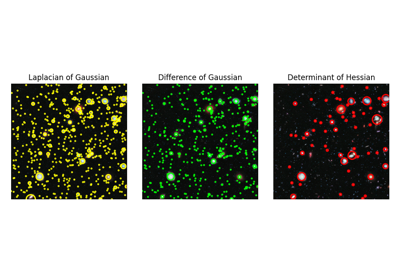## blob_doh¶

skimage.feature.blob_doh(image, min_sigma=1, max_sigma=30, num_sigma=10, threshold=0.01, overlap=0.5, log_scale=False, *, threshold_rel=None)[source]

Finds blobs in the given grayscale image.

Blobs are found using the Determinant of Hessian method . For each blob found, the method returns its coordinates and the standard deviation of the Gaussian Kernel used for the Hessian matrix whose determinant detected the blob. Determinant of Hessians is approximated using .

Parameters
image2D ndarray

Input grayscale image.Blobs can either be light on dark or vice versa.

min_sigmafloat, optional

The minimum standard deviation for Gaussian Kernel used to compute Hessian matrix. Keep this low to detect smaller blobs.

max_sigmafloat, optional

The maximum standard deviation for Gaussian Kernel used to compute Hessian matrix. Keep this high to detect larger blobs.

num_sigmaint, optional

The number of intermediate values of standard deviations to consider between min_sigma and max_sigma.

thresholdfloat or None, optional

The absolute lower bound for scale space maxima. Local maxima smaller than threshold are ignored. Reduce this to detect blobs with lower intensities. If threshold_rel is also specified, whichever threshold is larger will be used. If None, threshold_rel is used instead.

overlapfloat, optional

A value between 0 and 1. If the area of two blobs overlaps by a fraction greater than threshold, the smaller blob is eliminated.

log_scalebool, optional

If set intermediate values of standard deviations are interpolated using a logarithmic scale to the base 10. If not, linear interpolation is used.

threshold_relfloat or None, optional

Minimum intensity of peaks, calculated as max(doh_space) * threshold_rel, where doh_space refers to the stack of Determinant-of-Hessian (DoH) images computed internally. This should have a value between 0 and 1. If None, threshold is used instead.

Returns
A(n, 3) ndarray

A 2d array with each row representing 3 values, (y,x,sigma) where (y,x) are coordinates of the blob and sigma is the standard deviation of the Gaussian kernel of the Hessian Matrix whose determinant detected the blob.

Notes

The radius of each blob is approximately sigma. Computation of Determinant of Hessians is independent of the standard deviation. Therefore detecting larger blobs won’t take more time. In methods line blob_dog() and blob_log() the computation of Gaussians for larger sigma takes more time. The downside is that this method can’t be used for detecting blobs of radius less than 3px due to the box filters used in the approximation of Hessian Determinant.

References

1

https://en.wikipedia.org/wiki/Blob_detection#The_determinant_of_the_Hessian

2

Herbert Bay, Andreas Ess, Tinne Tuytelaars, Luc Van Gool, “SURF: Speeded Up Robust Features” ftp://ftp.vision.ee.ethz.ch/publications/articles/eth_biwi_00517.pdf

Examples

>>> from skimage import data, feature
>>> img = data.coins()
>>> feature.blob_doh(img)
array([[197.        , 153.        ,  20.33333333],
[124.        , 336.        ,  20.33333333],
[126.        , 153.        ,  20.33333333],
[195.        , 100.        ,  23.55555556],
[192.        , 212.        ,  23.55555556],
[121.        , 271.        ,  30.        ],
[126.        , 101.        ,  20.33333333],
[193.        , 275.        ,  23.55555556],
[123.        , 205.        ,  20.33333333],
[270.        , 363.        ,  30.        ],
[265.        , 113.        ,  23.55555556],
[262.        , 243.        ,  23.55555556],
[185.        , 348.        ,  30.        ],
[156.        , 302.        ,  30.        ],
[123.        ,  44.        ,  23.55555556],
[260.        , 173.        ,  30.        ],
[197.        ,  44.        ,  20.33333333]])


### Examples using skimage.feature.blob_doh¶## blob_log¶

skimage.feature.blob_log(image, min_sigma=1, max_sigma=50, num_sigma=10, threshold=0.2, overlap=0.5, log_scale=False, *, threshold_rel=None, exclude_border=False)[source]

Finds blobs in the given grayscale image.

Blobs are found using the Laplacian of Gaussian (LoG) method . For each blob found, the method returns its coordinates and the standard deviation of the Gaussian kernel that detected the blob.

Parameters
imagendarray

Input grayscale image, blobs are assumed to be light on dark background (white on black).

min_sigmascalar or sequence of scalars, optional

the minimum standard deviation for Gaussian kernel. Keep this low to detect smaller blobs. The standard deviations of the Gaussian filter are given for each axis as a sequence, or as a single number, in which case it is equal for all axes.

max_sigmascalar or sequence of scalars, optional

The maximum standard deviation for Gaussian kernel. Keep this high to detect larger blobs. The standard deviations of the Gaussian filter are given for each axis as a sequence, or as a single number, in which case it is equal for all axes.

num_sigmaint, optional

The number of intermediate values of standard deviations to consider between min_sigma and max_sigma.

thresholdfloat or None, optional

The absolute lower bound for scale space maxima. Local maxima smaller than threshold are ignored. Reduce this to detect blobs with lower intensities. If threshold_rel is also specified, whichever threshold is larger will be used. If None, threshold_rel is used instead.

overlapfloat, optional

A value between 0 and 1. If the area of two blobs overlaps by a fraction greater than threshold, the smaller blob is eliminated.

log_scalebool, optional

If set intermediate values of standard deviations are interpolated using a logarithmic scale to the base 10. If not, linear interpolation is used.

threshold_relfloat or None, optional

Minimum intensity of peaks, calculated as max(log_space) * threshold_rel, where log_space refers to the stack of Laplacian-of-Gaussian (LoG) images computed internally. This should have a value between 0 and 1. If None, threshold is used instead.

exclude_bordertuple of ints, int, or False, optional

If tuple of ints, the length of the tuple must match the input array’s dimensionality. Each element of the tuple will exclude peaks from within exclude_border-pixels of the border of the image along that dimension. If nonzero int, exclude_border excludes peaks from within exclude_border-pixels of the border of the image. If zero or False, peaks are identified regardless of their distance from the border.

Returns
A(n, image.ndim + sigma) ndarray

A 2d array with each row representing 2 coordinate values for a 2D image, or 3 coordinate values for a 3D image, plus the sigma(s) used. When a single sigma is passed, outputs are: (r, c, sigma) or (p, r, c, sigma) where (r, c) or (p, r, c) are coordinates of the blob and sigma is the standard deviation of the Gaussian kernel which detected the blob. When an anisotropic gaussian is used (sigmas per dimension), the detected sigma is returned for each dimension.

Notes

The radius of each blob is approximately $$\sqrt{2}\sigma$$ for a 2-D image and $$\sqrt{3}\sigma$$ for a 3-D image.

References

1

https://en.wikipedia.org/wiki/Blob_detection#The_Laplacian_of_Gaussian

Examples

>>> from skimage import data, feature, exposure
>>> img = data.coins()
>>> img = exposure.equalize_hist(img)  # improves detection
>>> feature.blob_log(img, threshold = .3)
array([[124.        , 336.        ,  11.88888889],
[198.        , 155.        ,  11.88888889],
[194.        , 213.        ,  17.33333333],
[121.        , 272.        ,  17.33333333],
[263.        , 244.        ,  17.33333333],
[194.        , 276.        ,  17.33333333],
[266.        , 115.        ,  11.88888889],
[128.        , 154.        ,  11.88888889],
[260.        , 174.        ,  17.33333333],
[198.        , 103.        ,  11.88888889],
[126.        , 208.        ,  11.88888889],
[127.        , 102.        ,  11.88888889],
[263.        , 302.        ,  17.33333333],
[197.        ,  44.        ,  11.88888889],
[185.        , 344.        ,  17.33333333],
[126.        ,  46.        ,  11.88888889],
[113.        , 323.        ,   1.        ]])


### Examples using skimage.feature.blob_log¶## canny¶

skimage.feature.canny(image, sigma=1.0, low_threshold=None, high_threshold=None, mask=None, use_quantiles=False, *, mode='constant', cval=0.0)[source]

Edge filter an image using the Canny algorithm.

Parameters
image2D array

Grayscale input image to detect edges on; can be of any dtype.

sigmafloat, optional

Standard deviation of the Gaussian filter.

low_thresholdfloat, optional

Lower bound for hysteresis thresholding (linking edges). If None, low_threshold is set to 10% of dtype’s max.

high_thresholdfloat, optional

Upper bound for hysteresis thresholding (linking edges). If None, high_threshold is set to 20% of dtype’s max.

Mask to limit the application of Canny to a certain area.

use_quantilesbool, optional

If True then treat low_threshold and high_threshold as quantiles of the edge magnitude image, rather than absolute edge magnitude values. If True then the thresholds must be in the range [0, 1].

modestr, {‘reflect’, ‘constant’, ‘nearest’, ‘mirror’, ‘wrap’}

The mode parameter determines how the array borders are handled during Gaussian filtering, where cval is the value when mode is equal to ‘constant’.

cvalfloat, optional

Value to fill past edges of input if mode is ‘constant’.

Returns
output2D array (image)

The binary edge map.

skimage.sobel

Notes

The steps of the algorithm are as follows:

• Smooth the image using a Gaussian with sigma width.

• Apply the horizontal and vertical Sobel operators to get the gradients within the image. The edge strength is the norm of the gradient.

• Thin potential edges to 1-pixel wide curves. First, find the normal to the edge at each point. This is done by looking at the signs and the relative magnitude of the X-Sobel and Y-Sobel to sort the points into 4 categories: horizontal, vertical, diagonal and antidiagonal. Then look in the normal and reverse directions to see if the values in either of those directions are greater than the point in question. Use interpolation to get a mix of points instead of picking the one that’s the closest to the normal.

• Perform a hysteresis thresholding: first label all points above the high threshold as edges. Then recursively label any point above the low threshold that is 8-connected to a labeled point as an edge.

References

1

Canny, J., A Computational Approach To Edge Detection, IEEE Trans. Pattern Analysis and Machine Intelligence, 8:679-714, 1986 DOI:10.1109/TPAMI.1986.4767851

2

William Green’s Canny tutorial https://en.wikipedia.org/wiki/Canny_edge_detector

Examples

>>> from skimage import feature
>>> rng = np.random.default_rng()
>>> # Generate noisy image of a square
>>> im = np.zeros((256, 256))
>>> im[64:-64, 64:-64] = 1
>>> im += 0.2 * rng.random(im.shape)
>>> # First trial with the Canny filter, with the default smoothing
>>> edges1 = feature.canny(im)
>>> # Increase the smoothing for better results
>>> edges2 = feature.canny(im, sigma=3)


### Examples using skimage.feature.canny¶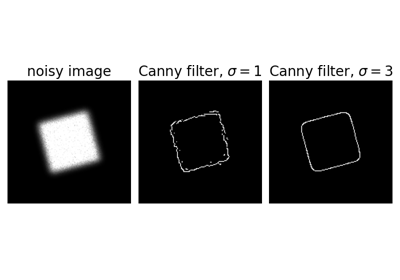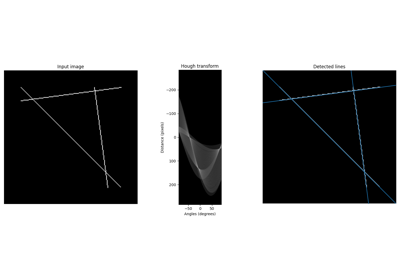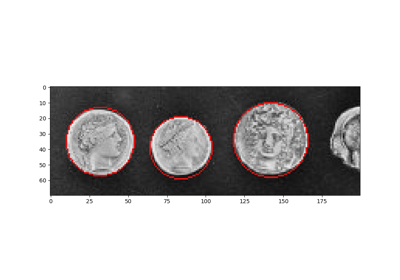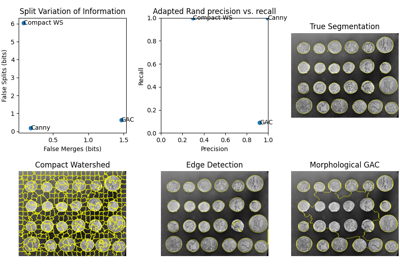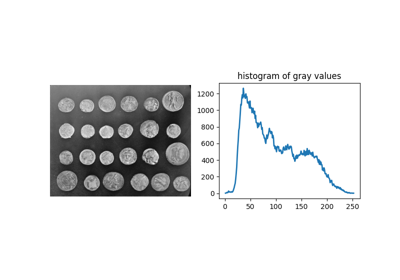## corner_fast¶

skimage.feature.corner_fast(image, n=12, threshold=0.15)[source]

Extract FAST corners for a given image.

Parameters
image(M, N) ndarray

Input image.

nint, optional

Minimum number of consecutive pixels out of 16 pixels on the circle that should all be either brighter or darker w.r.t testpixel. A point c on the circle is darker w.r.t test pixel p if Ic < Ip - threshold and brighter if Ic > Ip + threshold. Also stands for the n in FAST-n corner detector.

thresholdfloat, optional

Threshold used in deciding whether the pixels on the circle are brighter, darker or similar w.r.t. the test pixel. Decrease the threshold when more corners are desired and vice-versa.

Returns
responsendarray

FAST corner response image.

References

1

Rosten, E., & Drummond, T. (2006, May). Machine learning for high-speed corner detection. In European conference on computer vision (pp. 430-443). Springer, Berlin, Heidelberg. DOI:10.1007/11744023_34 http://www.edwardrosten.com/work/rosten_2006_machine.pdf

2

Wikipedia, “Features from accelerated segment test”, https://en.wikipedia.org/wiki/Features_from_accelerated_segment_test

Examples

>>> from skimage.feature import corner_fast, corner_peaks
>>> square = np.zeros((12, 12))
>>> square[3:9, 3:9] = 1
>>> square.astype(int)
array([[0, 0, 0, 0, 0, 0, 0, 0, 0, 0, 0, 0],
[0, 0, 0, 0, 0, 0, 0, 0, 0, 0, 0, 0],
[0, 0, 0, 0, 0, 0, 0, 0, 0, 0, 0, 0],
[0, 0, 0, 1, 1, 1, 1, 1, 1, 0, 0, 0],
[0, 0, 0, 1, 1, 1, 1, 1, 1, 0, 0, 0],
[0, 0, 0, 1, 1, 1, 1, 1, 1, 0, 0, 0],
[0, 0, 0, 1, 1, 1, 1, 1, 1, 0, 0, 0],
[0, 0, 0, 1, 1, 1, 1, 1, 1, 0, 0, 0],
[0, 0, 0, 1, 1, 1, 1, 1, 1, 0, 0, 0],
[0, 0, 0, 0, 0, 0, 0, 0, 0, 0, 0, 0],
[0, 0, 0, 0, 0, 0, 0, 0, 0, 0, 0, 0],
[0, 0, 0, 0, 0, 0, 0, 0, 0, 0, 0, 0]])
>>> corner_peaks(corner_fast(square, 9), min_distance=1)
array([[3, 3],
[3, 8],
[8, 3],
[8, 8]])


## corner_foerstner¶

skimage.feature.corner_foerstner(image, sigma=1)[source]

Compute Foerstner corner measure response image.

This corner detector uses information from the auto-correlation matrix A:

A = [(imx**2)   (imx*imy)] = [Axx Axy]
[(imx*imy)   (imy**2)]   [Axy Ayy]


Where imx and imy are first derivatives, averaged with a gaussian filter. The corner measure is then defined as:

w = det(A) / trace(A)           (size of error ellipse)
q = 4 * det(A) / trace(A)**2    (roundness of error ellipse)

Parameters
image(M, N) ndarray

Input image.

sigmafloat, optional

Standard deviation used for the Gaussian kernel, which is used as weighting function for the auto-correlation matrix.

Returns
wndarray

Error ellipse sizes.

qndarray

Roundness of error ellipse.

References

1

Förstner, W., & Gülch, E. (1987, June). A fast operator for detection and precise location of distinct points, corners and centres of circular features. In Proc. ISPRS intercommission conference on fast processing of photogrammetric data (pp. 281-305). https://cseweb.ucsd.edu/classes/sp02/cse252/foerstner/foerstner.pdf

2

https://en.wikipedia.org/wiki/Corner_detection

Examples

>>> from skimage.feature import corner_foerstner, corner_peaks
>>> square = np.zeros([10, 10])
>>> square[2:8, 2:8] = 1
>>> square.astype(int)
array([[0, 0, 0, 0, 0, 0, 0, 0, 0, 0],
[0, 0, 0, 0, 0, 0, 0, 0, 0, 0],
[0, 0, 1, 1, 1, 1, 1, 1, 0, 0],
[0, 0, 1, 1, 1, 1, 1, 1, 0, 0],
[0, 0, 1, 1, 1, 1, 1, 1, 0, 0],
[0, 0, 1, 1, 1, 1, 1, 1, 0, 0],
[0, 0, 1, 1, 1, 1, 1, 1, 0, 0],
[0, 0, 1, 1, 1, 1, 1, 1, 0, 0],
[0, 0, 0, 0, 0, 0, 0, 0, 0, 0],
[0, 0, 0, 0, 0, 0, 0, 0, 0, 0]])
>>> w, q = corner_foerstner(square)
>>> accuracy_thresh = 0.5
>>> roundness_thresh = 0.3
>>> foerstner = (q > roundness_thresh) * (w > accuracy_thresh) * w
>>> corner_peaks(foerstner, min_distance=1)
array([[2, 2],
[2, 7],
[7, 2],
[7, 7]])


## corner_harris¶

skimage.feature.corner_harris(image, method='k', k=0.05, eps=1e-06, sigma=1)[source]

Compute Harris corner measure response image.

This corner detector uses information from the auto-correlation matrix A:

A = [(imx**2)   (imx*imy)] = [Axx Axy]
[(imx*imy)   (imy**2)]   [Axy Ayy]


Where imx and imy are first derivatives, averaged with a gaussian filter. The corner measure is then defined as:

det(A) - k * trace(A)**2


or:

2 * det(A) / (trace(A) + eps)

Parameters
image(M, N) ndarray

Input image.

method{‘k’, ‘eps’}, optional

Method to compute the response image from the auto-correlation matrix.

kfloat, optional

Sensitivity factor to separate corners from edges, typically in range [0, 0.2]. Small values of k result in detection of sharp corners.

epsfloat, optional

Normalisation factor (Noble’s corner measure).

sigmafloat, optional

Standard deviation used for the Gaussian kernel, which is used as weighting function for the auto-correlation matrix.

Returns
responsendarray

Harris response image.

References

1

https://en.wikipedia.org/wiki/Corner_detection

Examples

>>> from skimage.feature import corner_harris, corner_peaks
>>> square = np.zeros([10, 10])
>>> square[2:8, 2:8] = 1
>>> square.astype(int)
array([[0, 0, 0, 0, 0, 0, 0, 0, 0, 0],
[0, 0, 0, 0, 0, 0, 0, 0, 0, 0],
[0, 0, 1, 1, 1, 1, 1, 1, 0, 0],
[0, 0, 1, 1, 1, 1, 1, 1, 0, 0],
[0, 0, 1, 1, 1, 1, 1, 1, 0, 0],
[0, 0, 1, 1, 1, 1, 1, 1, 0, 0],
[0, 0, 1, 1, 1, 1, 1, 1, 0, 0],
[0, 0, 1, 1, 1, 1, 1, 1, 0, 0],
[0, 0, 0, 0, 0, 0, 0, 0, 0, 0],
[0, 0, 0, 0, 0, 0, 0, 0, 0, 0]])
>>> corner_peaks(corner_harris(square), min_distance=1)
array([[2, 2],
[2, 7],
[7, 2],
[7, 7]])


### Examples using skimage.feature.corner_harris¶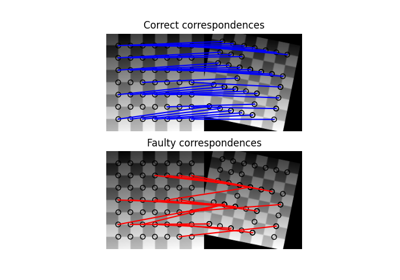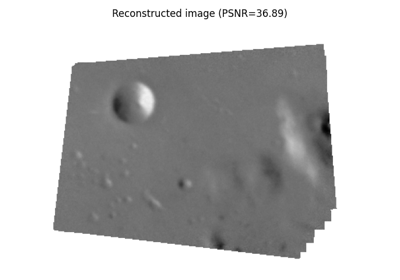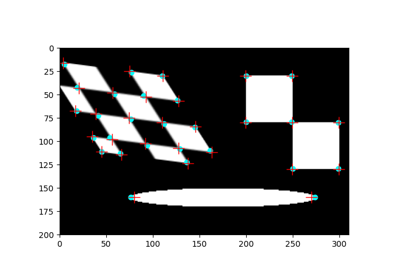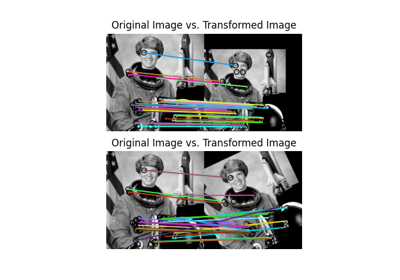## corner_kitchen_rosenfeld¶

skimage.feature.corner_kitchen_rosenfeld(image, mode='constant', cval=0)[source]

Compute Kitchen and Rosenfeld corner measure response image.

The corner measure is calculated as follows:

(imxx * imy**2 + imyy * imx**2 - 2 * imxy * imx * imy)
/ (imx**2 + imy**2)


Where imx and imy are the first and imxx, imxy, imyy the second derivatives.

Parameters
image(M, N) ndarray

Input image.

mode{‘constant’, ‘reflect’, ‘wrap’, ‘nearest’, ‘mirror’}, optional

How to handle values outside the image borders.

cvalfloat, optional

Used in conjunction with mode ‘constant’, the value outside the image boundaries.

Returns
responsendarray

Kitchen and Rosenfeld response image.

References

1

Kitchen, L., & Rosenfeld, A. (1982). Gray-level corner detection. Pattern recognition letters, 1(2), 95-102. DOI:10.1016/0167-8655(82)90020-4

## corner_moravec¶

skimage.feature.corner_moravec(image, window_size=1)[source]

Compute Moravec corner measure response image.

This is one of the simplest corner detectors and is comparatively fast but has several limitations (e.g. not rotation invariant).

Parameters
image(M, N) ndarray

Input image.

window_sizeint, optional

Window size.

Returns
responsendarray

Moravec response image.

References

1

https://en.wikipedia.org/wiki/Corner_detection

Examples

>>> from skimage.feature import corner_moravec
>>> square = np.zeros([7, 7])
>>> square[3, 3] = 1
>>> square.astype(int)
array([[0, 0, 0, 0, 0, 0, 0],
[0, 0, 0, 0, 0, 0, 0],
[0, 0, 0, 0, 0, 0, 0],
[0, 0, 0, 1, 0, 0, 0],
[0, 0, 0, 0, 0, 0, 0],
[0, 0, 0, 0, 0, 0, 0],
[0, 0, 0, 0, 0, 0, 0]])
>>> corner_moravec(square).astype(int)
array([[0, 0, 0, 0, 0, 0, 0],
[0, 0, 0, 0, 0, 0, 0],
[0, 0, 1, 1, 1, 0, 0],
[0, 0, 1, 2, 1, 0, 0],
[0, 0, 1, 1, 1, 0, 0],
[0, 0, 0, 0, 0, 0, 0],
[0, 0, 0, 0, 0, 0, 0]])


## corner_orientations¶

skimage.feature.corner_orientations(image, corners, mask)[source]

Compute the orientation of corners.

The orientation of corners is computed using the first order central moment i.e. the center of mass approach. The corner orientation is the angle of the vector from the corner coordinate to the intensity centroid in the local neighborhood around the corner calculated using first order central moment.

Parameters
image(M, N) array

Input grayscale image.

corners(K, 2) array

Corner coordinates as (row, col).

Mask defining the local neighborhood of the corner used for the calculation of the central moment.

Returns
orientations(K, 1) array

Orientations of corners in the range [-pi, pi].

References

1

Ethan Rublee, Vincent Rabaud, Kurt Konolige and Gary Bradski “ORB : An efficient alternative to SIFT and SURF” http://www.vision.cs.chubu.ac.jp/CV-R/pdf/Rublee_iccv2011.pdf

2

Paul L. Rosin, “Measuring Corner Properties” http://users.cs.cf.ac.uk/Paul.Rosin/corner2.pdf

Examples

>>> from skimage.morphology import octagon
>>> from skimage.feature import (corner_fast, corner_peaks,
...                              corner_orientations)
>>> square = np.zeros((12, 12))
>>> square[3:9, 3:9] = 1
>>> square.astype(int)
array([[0, 0, 0, 0, 0, 0, 0, 0, 0, 0, 0, 0],
[0, 0, 0, 0, 0, 0, 0, 0, 0, 0, 0, 0],
[0, 0, 0, 0, 0, 0, 0, 0, 0, 0, 0, 0],
[0, 0, 0, 1, 1, 1, 1, 1, 1, 0, 0, 0],
[0, 0, 0, 1, 1, 1, 1, 1, 1, 0, 0, 0],
[0, 0, 0, 1, 1, 1, 1, 1, 1, 0, 0, 0],
[0, 0, 0, 1, 1, 1, 1, 1, 1, 0, 0, 0],
[0, 0, 0, 1, 1, 1, 1, 1, 1, 0, 0, 0],
[0, 0, 0, 1, 1, 1, 1, 1, 1, 0, 0, 0],
[0, 0, 0, 0, 0, 0, 0, 0, 0, 0, 0, 0],
[0, 0, 0, 0, 0, 0, 0, 0, 0, 0, 0, 0],
[0, 0, 0, 0, 0, 0, 0, 0, 0, 0, 0, 0]])
>>> corners = corner_peaks(corner_fast(square, 9), min_distance=1)
>>> corners
array([[3, 3],
[3, 8],
[8, 3],
[8, 8]])
>>> orientations = corner_orientations(square, corners, octagon(3, 2))
array([  45.,  135.,  -45., -135.])


## corner_peaks¶

skimage.feature.corner_peaks(image, min_distance=1, threshold_abs=None, threshold_rel=None, exclude_border=True, indices=True, num_peaks=inf, footprint=None, labels=None, *, num_peaks_per_label=inf, p_norm=inf)[source]

Find peaks in corner measure response image.

This differs from skimage.feature.peak_local_max in that it suppresses multiple connected peaks with the same accumulator value.

Parameters
image(M, N) ndarray

Input image.

min_distanceint, optional

The minimal allowed distance separating peaks.

**
p_normfloat

Which Minkowski p-norm to use. Should be in the range [1, inf]. A finite large p may cause a ValueError if overflow can occur. inf corresponds to the Chebyshev distance and 2 to the Euclidean distance.

Returns
outputndarray or ndarray of bools
• If indices = True : (row, column, …) coordinates of peaks.

• If indices = False : Boolean array shaped like image, with peaks represented by True values.

Notes

Changed in version 0.18: The default value of threshold_rel has changed to None, which corresponds to letting skimage.feature.peak_local_max decide on the default. This is equivalent to threshold_rel=0.

The num_peaks limit is applied before suppression of connected peaks. To limit the number of peaks after suppression, set num_peaks=np.inf and post-process the output of this function.

Examples

>>> from skimage.feature import peak_local_max
>>> response = np.zeros((5, 5))
>>> response[2:4, 2:4] = 1
>>> response
array([[0., 0., 0., 0., 0.],
[0., 0., 0., 0., 0.],
[0., 0., 1., 1., 0.],
[0., 0., 1., 1., 0.],
[0., 0., 0., 0., 0.]])
>>> peak_local_max(response)
array([[2, 2],
[2, 3],
[3, 2],
[3, 3]])
>>> corner_peaks(response)
array([[2, 2]])


### Examples using skimage.feature.corner_peaks¶## corner_shi_tomasi¶

skimage.feature.corner_shi_tomasi(image, sigma=1)[source]

Compute Shi-Tomasi (Kanade-Tomasi) corner measure response image.

This corner detector uses information from the auto-correlation matrix A:

A = [(imx**2)   (imx*imy)] = [Axx Axy]
[(imx*imy)   (imy**2)]   [Axy Ayy]


Where imx and imy are first derivatives, averaged with a gaussian filter. The corner measure is then defined as the smaller eigenvalue of A:

((Axx + Ayy) - sqrt((Axx - Ayy)**2 + 4 * Axy**2)) / 2

Parameters
image(M, N) ndarray

Input image.

sigmafloat, optional

Standard deviation used for the Gaussian kernel, which is used as weighting function for the auto-correlation matrix.

Returns
responsendarray

Shi-Tomasi response image.

References

1

https://en.wikipedia.org/wiki/Corner_detection

Examples

>>> from skimage.feature import corner_shi_tomasi, corner_peaks
>>> square = np.zeros([10, 10])
>>> square[2:8, 2:8] = 1
>>> square.astype(int)
array([[0, 0, 0, 0, 0, 0, 0, 0, 0, 0],
[0, 0, 0, 0, 0, 0, 0, 0, 0, 0],
[0, 0, 1, 1, 1, 1, 1, 1, 0, 0],
[0, 0, 1, 1, 1, 1, 1, 1, 0, 0],
[0, 0, 1, 1, 1, 1, 1, 1, 0, 0],
[0, 0, 1, 1, 1, 1, 1, 1, 0, 0],
[0, 0, 1, 1, 1, 1, 1, 1, 0, 0],
[0, 0, 1, 1, 1, 1, 1, 1, 0, 0],
[0, 0, 0, 0, 0, 0, 0, 0, 0, 0],
[0, 0, 0, 0, 0, 0, 0, 0, 0, 0]])
>>> corner_peaks(corner_shi_tomasi(square), min_distance=1)
array([[2, 2],
[2, 7],
[7, 2],
[7, 7]])


## corner_subpix¶

skimage.feature.corner_subpix(image, corners, window_size=11, alpha=0.99)[source]

Determine subpixel position of corners.

A statistical test decides whether the corner is defined as the intersection of two edges or a single peak. Depending on the classification result, the subpixel corner location is determined based on the local covariance of the grey-values. If the significance level for either statistical test is not sufficient, the corner cannot be classified, and the output subpixel position is set to NaN.

Parameters
image(M, N) ndarray

Input image.

corners(K, 2) ndarray

Corner coordinates (row, col).

window_sizeint, optional

Search window size for subpixel estimation.

alphafloat, optional

Significance level for corner classification.

Returns
positions(K, 2) ndarray

Subpixel corner positions. NaN for “not classified” corners.

References

1

Förstner, W., & Gülch, E. (1987, June). A fast operator for detection and precise location of distinct points, corners and centres of circular features. In Proc. ISPRS intercommission conference on fast processing of photogrammetric data (pp. 281-305). https://cseweb.ucsd.edu/classes/sp02/cse252/foerstner/foerstner.pdf

2

https://en.wikipedia.org/wiki/Corner_detection

Examples

>>> from skimage.feature import corner_harris, corner_peaks, corner_subpix
>>> img = np.zeros((10, 10))
>>> img[:5, :5] = 1
>>> img[5:, 5:] = 1
>>> img.astype(int)
array([[1, 1, 1, 1, 1, 0, 0, 0, 0, 0],
[1, 1, 1, 1, 1, 0, 0, 0, 0, 0],
[1, 1, 1, 1, 1, 0, 0, 0, 0, 0],
[1, 1, 1, 1, 1, 0, 0, 0, 0, 0],
[1, 1, 1, 1, 1, 0, 0, 0, 0, 0],
[0, 0, 0, 0, 0, 1, 1, 1, 1, 1],
[0, 0, 0, 0, 0, 1, 1, 1, 1, 1],
[0, 0, 0, 0, 0, 1, 1, 1, 1, 1],
[0, 0, 0, 0, 0, 1, 1, 1, 1, 1],
[0, 0, 0, 0, 0, 1, 1, 1, 1, 1]])
>>> coords = corner_peaks(corner_harris(img), min_distance=2)
>>> coords_subpix = corner_subpix(img, coords, window_size=7)
>>> coords_subpix
array([[4.5, 4.5]])


### Examples using skimage.feature.corner_subpix¶## daisy¶

skimage.feature.daisy(image, step=4, radius=15, rings=3, histograms=8, orientations=8, normalization='l1', sigmas=None, ring_radii=None, visualize=False)[source]

Extract DAISY feature descriptors densely for the given image.

DAISY is a feature descriptor similar to SIFT formulated in a way that allows for fast dense extraction. Typically, this is practical for bag-of-features image representations.

The implementation follows Tola et al.  but deviate on the following points:

• Histogram bin contribution are smoothed with a circular Gaussian window over the tonal range (the angular range).

• The sigma values of the spatial Gaussian smoothing in this code do not match the sigma values in the original code by Tola et al. . In their code, spatial smoothing is applied to both the input image and the center histogram. However, this smoothing is not documented in  and, therefore, it is omitted.

Parameters
image(M, N) array

Input image (grayscale).

stepint, optional

Distance between descriptor sampling points.

Radius (in pixels) of the outermost ring.

ringsint, optional

Number of rings.

histogramsint, optional

Number of histograms sampled per ring.

orientationsint, optional

Number of orientations (bins) per histogram.

normalization[ ‘l1’ | ‘l2’ | ‘daisy’ | ‘off’ ], optional

How to normalize the descriptors

• ‘l1’: L1-normalization of each descriptor.

• ‘l2’: L2-normalization of each descriptor.

• ‘daisy’: L2-normalization of individual histograms.

• ‘off’: Disable normalization.

sigmas1D array of float, optional

Standard deviation of spatial Gaussian smoothing for the center histogram and for each ring of histograms. The array of sigmas should be sorted from the center and out. I.e. the first sigma value defines the spatial smoothing of the center histogram and the last sigma value defines the spatial smoothing of the outermost ring. Specifying sigmas overrides the following parameter.

rings = len(sigmas) - 1

Radius (in pixels) for each ring. Specifying ring_radii overrides the following two parameters.

rings = len(ring_radii) radius = ring_radii[-1]

If both sigmas and ring_radii are given, they must satisfy the following predicate since no radius is needed for the center histogram.

len(ring_radii) == len(sigmas) + 1

visualizebool, optional

Generate a visualization of the DAISY descriptors

Returns
descsarray

Grid of DAISY descriptors for the given image as an array dimensionality (P, Q, R) where

P = ceil((M - radius*2) / step) Q = ceil((N - radius*2) / step) R = (rings * histograms + 1) * orientations

descs_img(M, N, 3) array (only if visualize==True)

Visualization of the DAISY descriptors.

References

1(1,2)

Tola et al. “Daisy: An efficient dense descriptor applied to wide- baseline stereo.” Pattern Analysis and Machine Intelligence, IEEE Transactions on 32.5 (2010): 815-830.

2

http://cvlab.epfl.ch/software/daisy

### Examples using skimage.feature.daisy¶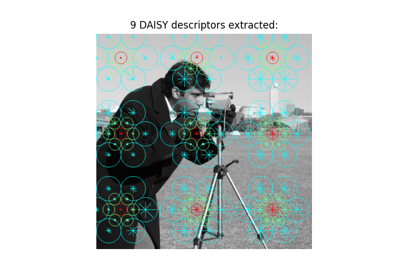## draw_haar_like_feature¶

skimage.feature.draw_haar_like_feature(image, r, c, width, height, feature_coord, color_positive_block=(1.0, 0.0, 0.0), color_negative_block=(0.0, 1.0, 0.0), alpha=0.5, max_n_features=None, random_state=None)[source]

Visualization of Haar-like features.

Parameters
image(M, N) ndarray

The region of an integral image for which the features need to be computed.

rint

Row-coordinate of top left corner of the detection window.

cint

Column-coordinate of top left corner of the detection window.

widthint

Width of the detection window.

heightint

Height of the detection window.

feature_coordndarray of list of tuples or None, optional

The array of coordinates to be extracted. This is useful when you want to recompute only a subset of features. In this case feature_type needs to be an array containing the type of each feature, as returned by haar_like_feature_coord(). By default, all coordinates are computed.

color_positive_blocktuple of 3 floats

Floats specifying the color for the positive block. Corresponding values define (R, G, B) values. Default value is red (1, 0, 0).

color_negative_blocktuple of 3 floats

Floats specifying the color for the negative block Corresponding values define (R, G, B) values. Default value is blue (0, 1, 0).

alphafloat

Value in the range [0, 1] that specifies opacity of visualization. 1 - fully transparent, 0 - opaque.

max_n_featuresint, default=None

The maximum number of features to be returned. By default, all features are returned.

random_state{None, int, numpy.random.Generator}, optional

If random_state is None the numpy.random.Generator singleton is used. If random_state is an int, a new Generator instance is used, seeded with random_state. If random_state is already a Generator instance then that instance is used.

The random state is used when generating a set of features smaller than the total number of available features.

Returns
features(M, N), ndarray

An image in which the different features will be added.

Examples

>>> import numpy as np
>>> from skimage.feature import haar_like_feature_coord
>>> from skimage.feature import draw_haar_like_feature
>>> feature_coord, _ = haar_like_feature_coord(2, 2, 'type-4')
>>> image = draw_haar_like_feature(np.zeros((2, 2)),
...                                0, 0, 2, 2,
...                                feature_coord,
...                                max_n_features=1)
>>> image
array([[[0. , 0.5, 0. ],
[0.5, 0. , 0. ]],

[[0.5, 0. , 0. ],
[0. , 0.5, 0. ]]])


### Examples using skimage.feature.draw_haar_like_feature¶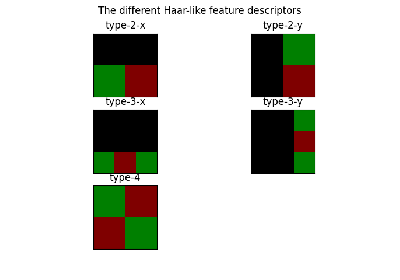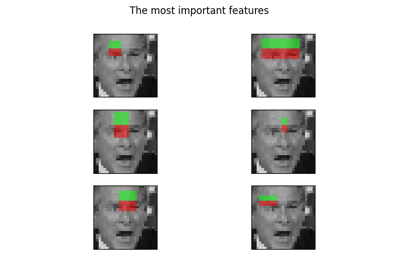## draw_multiblock_lbp¶

skimage.feature.draw_multiblock_lbp(image, r, c, width, height, lbp_code=0, color_greater_block=(1, 1, 1), color_less_block=(0, 0.69, 0.96), alpha=0.5)[source]

Multi-block local binary pattern visualization.

Blocks with higher sums are colored with alpha-blended white rectangles, whereas blocks with lower sums are colored alpha-blended cyan. Colors and the alpha parameter can be changed.

Parameters
imagendarray of float or uint

Image on which to visualize the pattern.

rint

Row-coordinate of top left corner of a rectangle containing feature.

cint

Column-coordinate of top left corner of a rectangle containing feature.

widthint

Width of one of 9 equal rectangles that will be used to compute a feature.

heightint

Height of one of 9 equal rectangles that will be used to compute a feature.

lbp_codeint

The descriptor of feature to visualize. If not provided, the descriptor with 0 value will be used.

color_greater_blocktuple of 3 floats

Floats specifying the color for the block that has greater intensity value. They should be in the range [0, 1]. Corresponding values define (R, G, B) values. Default value is white (1, 1, 1).

color_greater_blocktuple of 3 floats

Floats specifying the color for the block that has greater intensity value. They should be in the range [0, 1]. Corresponding values define (R, G, B) values. Default value is cyan (0, 0.69, 0.96).

alphafloat

Value in the range [0, 1] that specifies opacity of visualization. 1 - fully transparent, 0 - opaque.

Returns
outputndarray of float

Image with MB-LBP visualization.

References

1

L. Zhang, R. Chu, S. Xiang, S. Liao, S.Z. Li. “Face Detection Based on Multi-Block LBP Representation”, In Proceedings: Advances in Biometrics, International Conference, ICB 2007, Seoul, Korea. http://www.cbsr.ia.ac.cn/users/scliao/papers/Zhang-ICB07-MBLBP.pdf DOI:10.1007/978-3-540-74549-5_2

### Examples using skimage.feature.draw_multiblock_lbp¶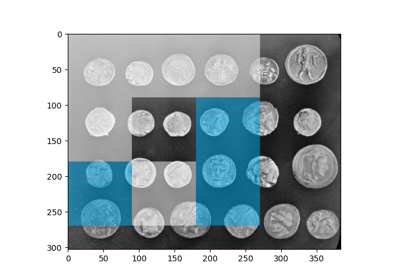## graycomatrix¶

skimage.feature.graycomatrix(image, distances, angles, levels=None, symmetric=False, normed=False)[source]

Calculate the gray-level co-occurrence matrix.

A gray level co-occurrence matrix is a histogram of co-occurring grayscale values at a given offset over an image.

Parameters
imagearray_like

Integer typed input image. Only positive valued images are supported. If type is other than uint8, the argument levels needs to be set.

distancesarray_like

List of pixel pair distance offsets.

anglesarray_like

List of pixel pair angles in radians.

levelsint, optional

The input image should contain integers in [0, levels-1], where levels indicate the number of gray-levels counted (typically 256 for an 8-bit image). This argument is required for 16-bit images or higher and is typically the maximum of the image. As the output matrix is at least levels x levels, it might be preferable to use binning of the input image rather than large values for levels.

symmetricbool, optional

If True, the output matrix P[:, :, d, theta] is symmetric. This is accomplished by ignoring the order of value pairs, so both (i, j) and (j, i) are accumulated when (i, j) is encountered for a given offset. The default is False.

normedbool, optional

If True, normalize each matrix P[:, :, d, theta] by dividing by the total number of accumulated co-occurrences for the given offset. The elements of the resulting matrix sum to 1. The default is False.

Returns
P4-D ndarray

The gray-level co-occurrence histogram. The value P[i,j,d,theta] is the number of times that gray-level j occurs at a distance d and at an angle theta from gray-level i. If normed is False, the output is of type uint32, otherwise it is float64. The dimensions are: levels x levels x number of distances x number of angles.

References

1

M. Hall-Beyer, 2007. GLCM Texture: A Tutorial https://prism.ucalgary.ca/handle/1880/51900 DOI:10.11575/PRISM/33280

2

R.M. Haralick, K. Shanmugam, and I. Dinstein, “Textural features for image classification”, IEEE Transactions on Systems, Man, and Cybernetics, vol. SMC-3, no. 6, pp. 610-621, Nov. 1973. DOI:10.1109/TSMC.1973.4309314

3

M. Nadler and E.P. Smith, Pattern Recognition Engineering, Wiley-Interscience, 1993.

4

Examples

Compute 2 GLCMs: One for a 1-pixel offset to the right, and one for a 1-pixel offset upwards.

>>> image = np.array([[0, 0, 1, 1],
...                   [0, 0, 1, 1],
...                   [0, 2, 2, 2],
...                   [2, 2, 3, 3]], dtype=np.uint8)
>>> result = graycomatrix(image, , [0, np.pi/4, np.pi/2, 3*np.pi/4],
...                       levels=4)
>>> result[:, :, 0, 0]
array([[2, 2, 1, 0],
[0, 2, 0, 0],
[0, 0, 3, 1],
[0, 0, 0, 1]], dtype=uint32)
>>> result[:, :, 0, 1]
array([[1, 1, 3, 0],
[0, 1, 1, 0],
[0, 0, 0, 2],
[0, 0, 0, 0]], dtype=uint32)
>>> result[:, :, 0, 2]
array([[3, 0, 2, 0],
[0, 2, 2, 0],
[0, 0, 1, 2],
[0, 0, 0, 0]], dtype=uint32)
>>> result[:, :, 0, 3]
array([[2, 0, 0, 0],
[1, 1, 2, 0],
[0, 0, 2, 1],
[0, 0, 0, 0]], dtype=uint32)


### Examples using skimage.feature.graycomatrix¶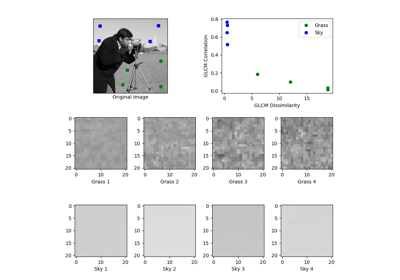## graycoprops¶

skimage.feature.graycoprops(P, prop='contrast')[source]

Calculate texture properties of a GLCM.

Compute a feature of a gray level co-occurrence matrix to serve as a compact summary of the matrix. The properties are computed as follows:

• ‘contrast’: $$\sum_{i,j=0}^{levels-1} P_{i,j}(i-j)^2$$

• ‘dissimilarity’: $$\sum_{i,j=0}^{levels-1}P_{i,j}|i-j|$$

• ‘homogeneity’: $$\sum_{i,j=0}^{levels-1}\frac{P_{i,j}}{1+(i-j)^2}$$

• ‘ASM’: $$\sum_{i,j=0}^{levels-1} P_{i,j}^2$$

• ‘energy’: $$\sqrt{ASM}$$

• ‘correlation’:
$\sum_{i,j=0}^{levels-1} P_{i,j}\left[\frac{(i-\mu_i) \ (j-\mu_j)}{\sqrt{(\sigma_i^2)(\sigma_j^2)}}\right]$

Each GLCM is normalized to have a sum of 1 before the computation of texture properties.

Parameters
Pndarray

Input array. P is the gray-level co-occurrence histogram for which to compute the specified property. The value P[i,j,d,theta] is the number of times that gray-level j occurs at a distance d and at an angle theta from gray-level i.

prop{‘contrast’, ‘dissimilarity’, ‘homogeneity’, ‘energy’, ‘correlation’, ‘ASM’}, optional

The property of the GLCM to compute. The default is ‘contrast’.

Returns
results2-D ndarray

2-dimensional array. results[d, a] is the property ‘prop’ for the d’th distance and the a’th angle.

References

1

M. Hall-Beyer, 2007. GLCM Texture: A Tutorial v. 1.0 through 3.0. The GLCM Tutorial Home Page, https://prism.ucalgary.ca/handle/1880/51900 DOI:10.11575/PRISM/33280

Examples

Compute the contrast for GLCMs with distances [1, 2] and angles [0 degrees, 90 degrees]

>>> image = np.array([[0, 0, 1, 1],
...                   [0, 0, 1, 1],
...                   [0, 2, 2, 2],
...                   [2, 2, 3, 3]], dtype=np.uint8)
>>> g = graycomatrix(image, [1, 2], [0, np.pi/2], levels=4,
...                  normed=True, symmetric=True)
>>> contrast = graycoprops(g, 'contrast')
>>> contrast
array([[0.58333333, 1.        ],
[1.25      , 2.75      ]])


### Examples using skimage.feature.graycoprops¶## greycomatrix¶

skimage.feature.greycomatrix(image, distances, angles, levels=None, symmetric=False, normed=False)[source]

Deprecated function. Use skimage.feature.graycomatrix instead.

## greycoprops¶

skimage.feature.greycoprops(P, prop='contrast')[source]

Deprecated function. Use skimage.feature.graycoprops instead.

## haar_like_feature¶

skimage.feature.haar_like_feature(int_image, r, c, width, height, feature_type=None, feature_coord=None)[source]

Compute the Haar-like features for a region of interest (ROI) of an integral image.

Haar-like features have been successfully used for image classification and object detection . It has been used for real-time face detection algorithm proposed in .

Parameters
int_image(M, N) ndarray

Integral image for which the features need to be computed.

rint

Row-coordinate of top left corner of the detection window.

cint

Column-coordinate of top left corner of the detection window.

widthint

Width of the detection window.

heightint

Height of the detection window.

feature_typestr or list of str or None, optional

The type of feature to consider:

• ‘type-2-x’: 2 rectangles varying along the x axis;

• ‘type-2-y’: 2 rectangles varying along the y axis;

• ‘type-3-x’: 3 rectangles varying along the x axis;

• ‘type-3-y’: 3 rectangles varying along the y axis;

• ‘type-4’: 4 rectangles varying along x and y axis.

By default all features are extracted.

If using with feature_coord, it should correspond to the feature type of each associated coordinate feature.

feature_coordndarray of list of tuples or None, optional

The array of coordinates to be extracted. This is useful when you want to recompute only a subset of features. In this case feature_type needs to be an array containing the type of each feature, as returned by haar_like_feature_coord(). By default, all coordinates are computed.

Returns
haar_features(n_features,) ndarray of int or float

Resulting Haar-like features. Each value is equal to the subtraction of sums of the positive and negative rectangles. The data type depends of the data type of int_image: int when the data type of int_image is uint or int and float when the data type of int_image is float.

Notes

When extracting those features in parallel, be aware that the choice of the backend (i.e. multiprocessing vs threading) will have an impact on the performance. The rule of thumb is as follows: use multiprocessing when extracting features for all possible ROI in an image; use threading when extracting the feature at specific location for a limited number of ROIs. Refer to the example Face classification using Haar-like feature descriptor for more insights.

References

1

https://en.wikipedia.org/wiki/Haar-like_feature

2

Oren, M., Papageorgiou, C., Sinha, P., Osuna, E., & Poggio, T. (1997, June). Pedestrian detection using wavelet templates. In Computer Vision and Pattern Recognition, 1997. Proceedings., 1997 IEEE Computer Society Conference on (pp. 193-199). IEEE. http://tinyurl.com/y6ulxfta DOI:10.1109/CVPR.1997.609319

3

Viola, Paul, and Michael J. Jones. “Robust real-time face detection.” International journal of computer vision 57.2 (2004): 137-154. https://www.merl.com/publications/docs/TR2004-043.pdf DOI:10.1109/CVPR.2001.990517

Examples

>>> import numpy as np
>>> from skimage.transform import integral_image
>>> from skimage.feature import haar_like_feature
>>> img = np.ones((5, 5), dtype=np.uint8)
>>> img_ii = integral_image(img)
>>> feature = haar_like_feature(img_ii, 0, 0, 5, 5, 'type-3-x')
>>> feature
array([-1, -2, -3, -4, -5, -1, -2, -3, -4, -5, -1, -2, -3, -4, -5, -1, -2,
-3, -4, -1, -2, -3, -4, -1, -2, -3, -4, -1, -2, -3, -1, -2, -3, -1,
-2, -3, -1, -2, -1, -2, -1, -2, -1, -1, -1])


You can compute the feature for some pre-computed coordinates.

>>> from skimage.feature import haar_like_feature_coord
>>> feature_coord, feature_type = zip(
...     *[haar_like_feature_coord(5, 5, feat_t)
...       for feat_t in ('type-2-x', 'type-3-x')])
>>> # only select one feature over two
>>> feature_coord = np.concatenate([x[::2] for x in feature_coord])
>>> feature_type = np.concatenate([x[::2] for x in feature_type])
>>> feature = haar_like_feature(img_ii, 0, 0, 5, 5,
...                             feature_type=feature_type,
...                             feature_coord=feature_coord)
>>> feature
array([ 0,  0,  0,  0,  0,  0,  0,  0,  0,  0,  0,  0,  0,  0,  0,  0,  0,
0,  0,  0,  0,  0,  0,  0,  0,  0,  0,  0,  0,  0,  0,  0,  0,  0,
0,  0,  0,  0,  0,  0,  0,  0,  0,  0,  0, -1, -3, -5, -2, -4, -1,
-3, -5, -2, -4, -2, -4, -2, -4, -2, -1, -3, -2, -1, -1, -1, -1, -1])


### Examples using skimage.feature.haar_like_feature¶## haar_like_feature_coord¶

skimage.feature.haar_like_feature_coord(width, height, feature_type=None)[source]

Compute the coordinates of Haar-like features.

Parameters
widthint

Width of the detection window.

heightint

Height of the detection window.

feature_typestr or list of str or None, optional

The type of feature to consider:

• ‘type-2-x’: 2 rectangles varying along the x axis;

• ‘type-2-y’: 2 rectangles varying along the y axis;

• ‘type-3-x’: 3 rectangles varying along the x axis;

• ‘type-3-y’: 3 rectangles varying along the y axis;

• ‘type-4’: 4 rectangles varying along x and y axis.

By default all features are extracted.

Returns
feature_coord(n_features, n_rectangles, 2, 2), ndarray of list of tuple coord

Coordinates of the rectangles for each feature.

feature_type(n_features,), ndarray of str

The corresponding type for each feature.

Examples

>>> import numpy as np
>>> from skimage.transform import integral_image
>>> from skimage.feature import haar_like_feature_coord
>>> feat_coord, feat_type = haar_like_feature_coord(2, 2, 'type-4')
>>> feat_coord
array([ list([[(0, 0), (0, 0)], [(0, 1), (0, 1)],
[(1, 1), (1, 1)], [(1, 0), (1, 0)]])], dtype=object)
>>> feat_type
array(['type-4'], dtype=object)


### Examples using skimage.feature.haar_like_feature_coord¶## hessian_matrix¶

skimage.feature.hessian_matrix(image, sigma=1, mode='constant', cval=0, order='rc')[source]

Compute the Hessian matrix.

In 2D, the Hessian matrix is defined as:

H = [Hrr Hrc]
[Hrc Hcc]


which is computed by convolving the image with the second derivatives of the Gaussian kernel in the respective r- and c-directions.

The implementation here also supports n-dimensional data.

Parameters
imagendarray

Input image.

sigmafloat

Standard deviation used for the Gaussian kernel, which is used as weighting function for the auto-correlation matrix.

mode{‘constant’, ‘reflect’, ‘wrap’, ‘nearest’, ‘mirror’}, optional

How to handle values outside the image borders.

cvalfloat, optional

Used in conjunction with mode ‘constant’, the value outside the image boundaries.

order{‘rc’, ‘xy’}, optional

This parameter allows for the use of reverse or forward order of the image axes in gradient computation. ‘rc’ indicates the use of the first axis initially (Hrr, Hrc, Hcc), whilst ‘xy’ indicates the usage of the last axis initially (Hxx, Hxy, Hyy)

Returns
H_elemslist of ndarray

Upper-diagonal elements of the hessian matrix for each pixel in the input image. In 2D, this will be a three element list containing [Hrr, Hrc, Hcc]. In nD, the list will contain (n**2 + n) / 2 arrays.

Examples

>>> from skimage.feature import hessian_matrix
>>> square = np.zeros((5, 5))
>>> square[2, 2] = 4
>>> Hrr, Hrc, Hcc = hessian_matrix(square, sigma=0.1, order='rc')
>>> Hrc
array([[ 0.,  0.,  0.,  0.,  0.],
[ 0.,  1.,  0., -1.,  0.],
[ 0.,  0.,  0.,  0.,  0.],
[ 0., -1.,  0.,  1.,  0.],
[ 0.,  0.,  0.,  0.,  0.]])


## hessian_matrix_det¶

skimage.feature.hessian_matrix_det(image, sigma=1, approximate=True)[source]

Compute the approximate Hessian Determinant over an image.

The 2D approximate method uses box filters over integral images to compute the approximate Hessian Determinant.

Parameters
imagendarray

The image over which to compute the Hessian Determinant.

sigmafloat, optional

Standard deviation of the Gaussian kernel used for the Hessian matrix.

approximatebool, optional

If True and the image is 2D, use a much faster approximate computation. This argument has no effect on 3D and higher images.

Returns
outarray

The array of the Determinant of Hessians.

Notes

For 2D images when approximate=True, the running time of this method only depends on size of the image. It is independent of sigma as one would expect. The downside is that the result for sigma less than 3 is not accurate, i.e., not similar to the result obtained if someone computed the Hessian and took its determinant.

References

1

Herbert Bay, Andreas Ess, Tinne Tuytelaars, Luc Van Gool, “SURF: Speeded Up Robust Features” ftp://ftp.vision.ee.ethz.ch/publications/articles/eth_biwi_00517.pdf

## hessian_matrix_eigvals¶

skimage.feature.hessian_matrix_eigvals(H_elems)[source]

Compute eigenvalues of Hessian matrix.

Parameters
H_elemslist of ndarray

The upper-diagonal elements of the Hessian matrix, as returned by hessian_matrix.

Returns
eigsndarray

The eigenvalues of the Hessian matrix, in decreasing order. The eigenvalues are the leading dimension. That is, eigs[i, j, k] contains the ith-largest eigenvalue at position (j, k).

Examples

>>> from skimage.feature import hessian_matrix, hessian_matrix_eigvals
>>> square = np.zeros((5, 5))
>>> square[2, 2] = 4
>>> H_elems = hessian_matrix(square, sigma=0.1, order='rc')
>>> hessian_matrix_eigvals(H_elems)
array([[ 0.,  0.,  2.,  0.,  0.],
[ 0.,  1.,  0.,  1.,  0.],
[ 2.,  0., -2.,  0.,  2.],
[ 0.,  1.,  0.,  1.,  0.],
[ 0.,  0.,  2.,  0.,  0.]])


## hog¶

skimage.feature.hog(image, orientations=9, pixels_per_cell=(8, 8), cells_per_block=(3, 3), block_norm='L2-Hys', visualize=False, transform_sqrt=False, feature_vector=True, multichannel=None, *, channel_axis=None)[source]

Extract Histogram of Oriented Gradients (HOG) for a given image.

Compute a Histogram of Oriented Gradients (HOG) by

1. (optional) global image normalization

2. computing the gradient image in row and col

4. normalizing across blocks

5. flattening into a feature vector

Parameters
image(M, N[, C]) ndarray

Input image.

orientationsint, optional

Number of orientation bins.

pixels_per_cell2-tuple (int, int), optional

Size (in pixels) of a cell.

cells_per_block2-tuple (int, int), optional

Number of cells in each block.

block_normstr {‘L1’, ‘L1-sqrt’, ‘L2’, ‘L2-Hys’}, optional

Block normalization method:

L1

Normalization using L1-norm.

L1-sqrt

Normalization using L1-norm, followed by square root.

L2

Normalization using L2-norm.

L2-Hys

Normalization using L2-norm, followed by limiting the maximum values to 0.2 (Hys stands for hysteresis) and renormalization using L2-norm. (default) For details, see , .

visualizebool, optional

Also return an image of the HOG. For each cell and orientation bin, the image contains a line segment that is centered at the cell center, is perpendicular to the midpoint of the range of angles spanned by the orientation bin, and has intensity proportional to the corresponding histogram value.

transform_sqrtbool, optional

Apply power law compression to normalize the image before processing. DO NOT use this if the image contains negative values. Also see notes section below.

feature_vectorbool, optional

Return the data as a feature vector by calling .ravel() on the result just before returning.

multichannelboolean, optional

If True, the last image dimension is considered as a color channel, otherwise as spatial. This argument is deprecated: specify channel_axis instead.

channel_axisint or None, optional

If None, the image is assumed to be a grayscale (single channel) image. Otherwise, this parameter indicates which axis of the array corresponds to channels.

New in version 0.19: channel_axis was added in 0.19.

Returns
out(n_blocks_row, n_blocks_col, n_cells_row, n_cells_col, n_orient) ndarray

HOG descriptor for the image. If feature_vector is True, a 1D (flattened) array is returned.

hog_image(M, N) ndarray, optional

A visualisation of the HOG image. Only provided if visualize is True.

Other Parameters
multichannelDEPRECATED

Deprecated in favor of channel_axis.

Deprecated since version 0.19.

Notes

The presented code implements the HOG extraction method from  with the following changes: (I) blocks of (3, 3) cells are used ((2, 2) in the paper); (II) no smoothing within cells (Gaussian spatial window with sigma=8pix in the paper); (III) L1 block normalization is used (L2-Hys in the paper).

Power law compression, also known as Gamma correction, is used to reduce the effects of shadowing and illumination variations. The compression makes the dark regions lighter. When the kwarg transform_sqrt is set to True, the function computes the square root of each color channel and then applies the hog algorithm to the image.

References

1

2

Dalal, N and Triggs, B, Histograms of Oriented Gradients for Human Detection, IEEE Computer Society Conference on Computer Vision and Pattern Recognition 2005 San Diego, CA, USA, https://lear.inrialpes.fr/people/triggs/pubs/Dalal-cvpr05.pdf, DOI:10.1109/CVPR.2005.177

3

Lowe, D.G., Distinctive image features from scale-invatiant keypoints, International Journal of Computer Vision (2004) 60: 91, http://www.cs.ubc.ca/~lowe/papers/ijcv04.pdf, DOI:10.1023/B:VISI.0000029664.99615.94

4

Dalal, N, Finding People in Images and Videos, Human-Computer Interaction [cs.HC], Institut National Polytechnique de Grenoble - INPG, 2006, https://tel.archives-ouvertes.fr/tel-00390303/file/NavneetDalalThesis.pdf

### Examples using skimage.feature.hog¶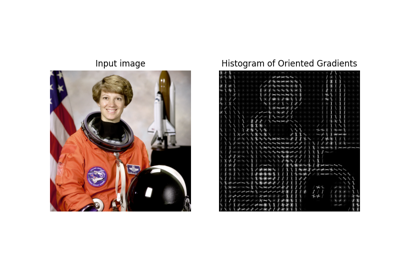## local_binary_pattern¶

skimage.feature.local_binary_pattern(image, P, R, method='default')[source]

Gray scale and rotation invariant LBP (Local Binary Patterns).

LBP is an invariant descriptor that can be used for texture classification.

Parameters
image(N, M) array

Graylevel image.

Pint

Number of circularly symmetric neighbour set points (quantization of the angular space).

Rfloat

Radius of circle (spatial resolution of the operator).

method{‘default’, ‘ror’, ‘uniform’, ‘var’}

Method to determine the pattern.

• ‘default’: original local binary pattern which is gray scale but not

rotation invariant.

• ‘ror’: extension of default implementation which is gray scale and

rotation invariant.

• ‘uniform’: improved rotation invariance with uniform patterns and

finer quantization of the angular space which is gray scale and rotation invariant.

• ‘nri_uniform’: non rotation-invariant uniform patterns variant

which is only gray scale invariant , .

• ‘var’: rotation invariant variance measures of the contrast of local

image texture which is rotation but not gray scale invariant.

Returns
output(N, M) array

LBP image.

References

1

T. Ojala, M. Pietikainen, T. Maenpaa, “Multiresolution gray-scale and rotation invariant texture classification with local binary patterns”, IEEE Transactions on Pattern Analysis and Machine Intelligence, vol. 24, no. 7, pp. 971-987, July 2002 DOI:10.1109/TPAMI.2002.1017623

2

T. Ahonen, A. Hadid and M. Pietikainen. “Face recognition with local binary patterns”, in Proc. Eighth European Conf. Computer Vision, Prague, Czech Republic, May 11-14, 2004, pp. 469-481, 2004. http://citeseerx.ist.psu.edu/viewdoc/summary?doi=10.1.1.214.6851 DOI:10.1007/978-3-540-24670-1_36

3

T. Ahonen, A. Hadid and M. Pietikainen, “Face Description with Local Binary Patterns: Application to Face Recognition”, IEEE Transactions on Pattern Analysis and Machine Intelligence, vol. 28, no. 12, pp. 2037-2041, Dec. 2006 DOI:10.1109/TPAMI.2006.244.

### Examples using skimage.feature.local_binary_pattern¶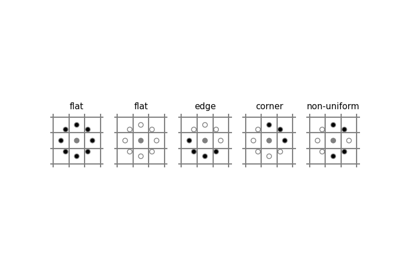## match_descriptors¶

skimage.feature.match_descriptors(descriptors1, descriptors2, metric=None, p=2, max_distance=inf, cross_check=True, max_ratio=1.0)[source]

Brute-force matching of descriptors.

For each descriptor in the first set this matcher finds the closest descriptor in the second set (and vice-versa in the case of enabled cross-checking).

Parameters
descriptors1(M, P) array

Descriptors of size P about M keypoints in the first image.

descriptors2(N, P) array

Descriptors of size P about N keypoints in the second image.

metric{‘euclidean’, ‘cityblock’, ‘minkowski’, ‘hamming’, …} , optional

The metric to compute the distance between two descriptors. See scipy.spatial.distance.cdist for all possible types. The hamming distance should be used for binary descriptors. By default the L2-norm is used for all descriptors of dtype float or double and the Hamming distance is used for binary descriptors automatically.

pint, optional

The p-norm to apply for metric='minkowski'.

max_distancefloat, optional

Maximum allowed distance between descriptors of two keypoints in separate images to be regarded as a match.

cross_checkbool, optional

If True, the matched keypoints are returned after cross checking i.e. a matched pair (keypoint1, keypoint2) is returned if keypoint2 is the best match for keypoint1 in second image and keypoint1 is the best match for keypoint2 in first image.

max_ratiofloat, optional

Maximum ratio of distances between first and second closest descriptor in the second set of descriptors. This threshold is useful to filter ambiguous matches between the two descriptor sets. The choice of this value depends on the statistics of the chosen descriptor, e.g., for SIFT descriptors a value of 0.8 is usually chosen, see D.G. Lowe, “Distinctive Image Features from Scale-Invariant Keypoints”, International Journal of Computer Vision, 2004.

Returns
matches(Q, 2) array

Indices of corresponding matches in first and second set of descriptors, where matches[:, 0] denote the indices in the first and matches[:, 1] the indices in the second set of descriptors.

### Examples using skimage.feature.match_descriptors¶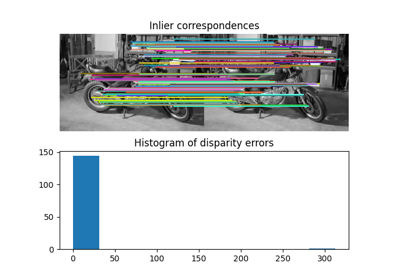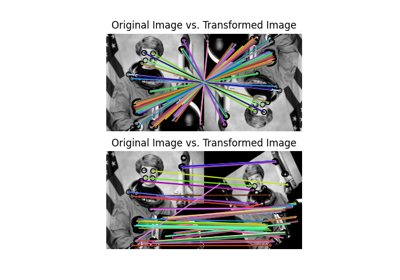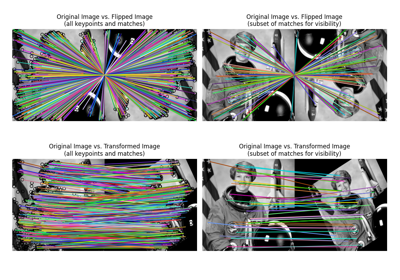## match_template¶

skimage.feature.match_template(image, template, pad_input=False, mode='constant', constant_values=0)[source]

Match a template to a 2-D or 3-D image using normalized correlation.

The output is an array with values between -1.0 and 1.0. The value at a given position corresponds to the correlation coefficient between the image and the template.

For pad_input=True matches correspond to the center and otherwise to the top-left corner of the template. To find the best match you must search for peaks in the response (output) image.

Parameters
image(M, N[, D]) array

2-D or 3-D input image.

template(m, n[, d]) array

Template to locate. It must be (m <= M, n <= N[, d <= D]).

If True, pad image so that output is the same size as the image, and output values correspond to the template center. Otherwise, the output is an array with shape (M - m + 1, N - n + 1) for an (M, N) image and an (m, n) template, and matches correspond to origin (top-left corner) of the template.

modesee numpy.pad, optional

constant_valuessee numpy.pad, optional

Constant values used in conjunction with mode='constant'.

Returns
outputarray

Response image with correlation coefficients.

Notes

Details on the cross-correlation are presented in . This implementation uses FFT convolutions of the image and the template. Reference  presents similar derivations but the approximation presented in this reference is not used in our implementation.

References

1

J. P. Lewis, “Fast Normalized Cross-Correlation”, Industrial Light and Magic.

2

Briechle and Hanebeck, “Template Matching using Fast Normalized Cross Correlation”, Proceedings of the SPIE (2001). DOI:10.1117/12.421129

Examples

>>> template = np.zeros((3, 3))
>>> template[1, 1] = 1
>>> template
array([[0., 0., 0.],
[0., 1., 0.],
[0., 0., 0.]])
>>> image = np.zeros((6, 6))
>>> image[1, 1] = 1
>>> image[4, 4] = -1
>>> image
array([[ 0.,  0.,  0.,  0.,  0.,  0.],
[ 0.,  1.,  0.,  0.,  0.,  0.],
[ 0.,  0.,  0.,  0.,  0.,  0.],
[ 0.,  0.,  0.,  0.,  0.,  0.],
[ 0.,  0.,  0.,  0., -1.,  0.],
[ 0.,  0.,  0.,  0.,  0.,  0.]])
>>> result = match_template(image, template)
>>> np.round(result, 3)
array([[ 1.   , -0.125,  0.   ,  0.   ],
[-0.125, -0.125,  0.   ,  0.   ],
[ 0.   ,  0.   ,  0.125,  0.125],
[ 0.   ,  0.   ,  0.125, -1.   ]])
>>> result = match_template(image, template, pad_input=True)
>>> np.round(result, 3)
array([[-0.125, -0.125, -0.125,  0.   ,  0.   ,  0.   ],
[-0.125,  1.   , -0.125,  0.   ,  0.   ,  0.   ],
[-0.125, -0.125, -0.125,  0.   ,  0.   ,  0.   ],
[ 0.   ,  0.   ,  0.   ,  0.125,  0.125,  0.125],
[ 0.   ,  0.   ,  0.   ,  0.125, -1.   ,  0.125],
[ 0.   ,  0.   ,  0.   ,  0.125,  0.125,  0.125]])


### Examples using skimage.feature.match_template¶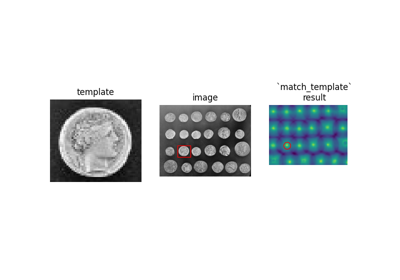## multiblock_lbp¶

skimage.feature.multiblock_lbp(int_image, r, c, width, height)[source]

Multi-block local binary pattern (MB-LBP).

The features are calculated similarly to local binary patterns (LBPs), (See local_binary_pattern()) except that summed blocks are used instead of individual pixel values.

MB-LBP is an extension of LBP that can be computed on multiple scales in constant time using the integral image. Nine equally-sized rectangles are used to compute a feature. For each rectangle, the sum of the pixel intensities is computed. Comparisons of these sums to that of the central rectangle determine the feature, similarly to LBP.

Parameters
int_image(N, M) array

Integral image.

rint

Row-coordinate of top left corner of a rectangle containing feature.

cint

Column-coordinate of top left corner of a rectangle containing feature.

widthint

Width of one of the 9 equal rectangles that will be used to compute a feature.

heightint

Height of one of the 9 equal rectangles that will be used to compute a feature.

Returns
outputint

8-bit MB-LBP feature descriptor.

References

1

L. Zhang, R. Chu, S. Xiang, S. Liao, S.Z. Li. “Face Detection Based on Multi-Block LBP Representation”, In Proceedings: Advances in Biometrics, International Conference, ICB 2007, Seoul, Korea. http://www.cbsr.ia.ac.cn/users/scliao/papers/Zhang-ICB07-MBLBP.pdf DOI:10.1007/978-3-540-74549-5_2

### Examples using skimage.feature.multiblock_lbp¶## multiscale_basic_features¶

skimage.feature.multiscale_basic_features(image, multichannel=False, intensity=True, edges=True, texture=True, sigma_min=0.5, sigma_max=16, num_sigma=None, num_workers=None, *, channel_axis=None)[source]

Local features for a single- or multi-channel nd image.

Intensity, gradient intensity and local structure are computed at different scales thanks to Gaussian blurring.

Parameters
imagendarray

Input image, which can be grayscale or multichannel.

multichannelbool, default False

True if the last dimension corresponds to color channels. This argument is deprecated: specify channel_axis instead.

intensitybool, default True

If True, pixel intensities averaged over the different scales are added to the feature set.

edgesbool, default True

If True, intensities of local gradients averaged over the different scales are added to the feature set.

texturebool, default True

If True, eigenvalues of the Hessian matrix after Gaussian blurring at different scales are added to the feature set.

sigma_minfloat, optional

Smallest value of the Gaussian kernel used to average local neighbourhoods before extracting features.

sigma_maxfloat, optional

Largest value of the Gaussian kernel used to average local neighbourhoods before extracting features.

num_sigmaint, optional

Number of values of the Gaussian kernel between sigma_min and sigma_max. If None, sigma_min multiplied by powers of 2 are used.

num_workersint or None, optional

The number of parallel threads to use. If set to None, the full set of available cores are used.

channel_axisint or None, optional

If None, the image is assumed to be a grayscale (single channel) image. Otherwise, this parameter indicates which axis of the array corresponds to channels.

New in version 0.19: channel_axis was added in 0.19.

Returns
featuresnp.ndarray

Array of shape image.shape + (n_features,). When channel_axis is not None, all channels are concatenated along the features dimension. (i.e. n_features == n_features_singlechannel * n_channels)

Other Parameters
multichannelDEPRECATED

Deprecated in favor of channel_axis.

Deprecated since version 0.19.

### Examples using skimage.feature.multiscale_basic_features¶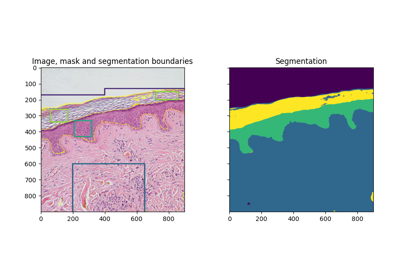## peak_local_max¶

skimage.feature.peak_local_max(image, min_distance=1, threshold_abs=None, threshold_rel=None, exclude_border=True, indices=True, num_peaks=inf, footprint=None, labels=None, num_peaks_per_label=inf, p_norm=inf)[source]

Find peaks in an image as coordinate list or boolean mask.

Peaks are the local maxima in a region of 2 * min_distance + 1 (i.e. peaks are separated by at least min_distance).

If both threshold_abs and threshold_rel are provided, the maximum of the two is chosen as the minimum intensity threshold of peaks.

Changed in version 0.18: Prior to version 0.18, peaks of the same height within a radius of min_distance were all returned, but this could cause unexpected behaviour. From 0.18 onwards, an arbitrary peak within the region is returned. See issue gh-2592.

Parameters
imagendarray

Input image.

min_distanceint, optional

The minimal allowed distance separating peaks. To find the maximum number of peaks, use min_distance=1.

threshold_absfloat or None, optional

Minimum intensity of peaks. By default, the absolute threshold is the minimum intensity of the image.

threshold_relfloat or None, optional

Minimum intensity of peaks, calculated as max(image) * threshold_rel.

exclude_borderint, tuple of ints, or bool, optional

If positive integer, exclude_border excludes peaks from within exclude_border-pixels of the border of the image. If tuple of non-negative ints, the length of the tuple must match the input array’s dimensionality. Each element of the tuple will exclude peaks from within exclude_border-pixels of the border of the image along that dimension. If True, takes the min_distance parameter as value. If zero or False, peaks are identified regardless of their distance from the border.

indicesbool, optional

If True, the output will be an array representing peak coordinates. The coordinates are sorted according to peaks values (Larger first). If False, the output will be a boolean array shaped as image.shape with peaks present at True elements. indices is deprecated and will be removed in version 0.20. Default behavior will be to always return peak coordinates. You can obtain a mask as shown in the example below.

num_peaksint, optional

Maximum number of peaks. When the number of peaks exceeds num_peaks, return num_peaks peaks based on highest peak intensity.

footprintndarray of bools, optional

If provided, footprint == 1 represents the local region within which to search for peaks at every point in image.

labelsndarray of ints, optional

If provided, each unique region labels == value represents a unique region to search for peaks. Zero is reserved for background.

num_peaks_per_labelint, optional

Maximum number of peaks for each label.

p_normfloat

Which Minkowski p-norm to use. Should be in the range [1, inf]. A finite large p may cause a ValueError if overflow can occur. inf corresponds to the Chebyshev distance and 2 to the Euclidean distance.

Returns
outputndarray or ndarray of bools
• If indices = True : (row, column, …) coordinates of peaks.

• If indices = False : Boolean array shaped like image, with peaks represented by True values.

Notes

The peak local maximum function returns the coordinates of local peaks (maxima) in an image. Internally, a maximum filter is used for finding local maxima. This operation dilates the original image. After comparison of the dilated and original image, this function returns the coordinates or a mask of the peaks where the dilated image equals the original image.

Examples

>>> img1 = np.zeros((7, 7))
>>> img1[3, 4] = 1
>>> img1[3, 2] = 1.5
>>> img1
array([[0. , 0. , 0. , 0. , 0. , 0. , 0. ],
[0. , 0. , 0. , 0. , 0. , 0. , 0. ],
[0. , 0. , 0. , 0. , 0. , 0. , 0. ],
[0. , 0. , 1.5, 0. , 1. , 0. , 0. ],
[0. , 0. , 0. , 0. , 0. , 0. , 0. ],
[0. , 0. , 0. , 0. , 0. , 0. , 0. ],
[0. , 0. , 0. , 0. , 0. , 0. , 0. ]])

>>> peak_local_max(img1, min_distance=1)
array([[3, 2],
[3, 4]])

>>> peak_local_max(img1, min_distance=2)
array([[3, 2]])

>>> img2 = np.zeros((20, 20, 20))
>>> img2[10, 10, 10] = 1
>>> img2[15, 15, 15] = 1
>>> peak_idx = peak_local_max(img2, exclude_border=0)
>>> peak_idx
array([[10, 10, 10],
[15, 15, 15]])

>>> peak_mask = np.zeros_like(img2, dtype=bool)
array([[10, 10, 10],
[15, 15, 15]])


### Examples using skimage.feature.peak_local_max¶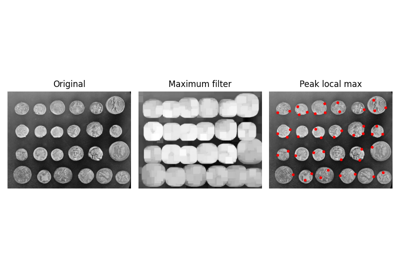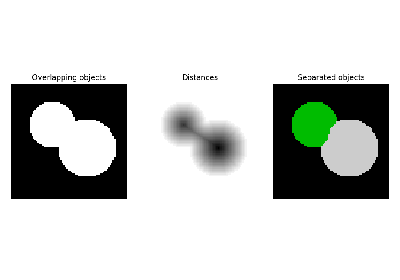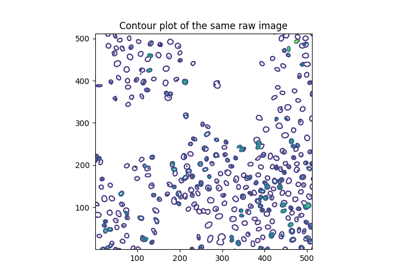## plot_matches¶

skimage.feature.plot_matches(ax, image1, image2, keypoints1, keypoints2, matches, keypoints_color='k', matches_color=None, only_matches=False, alignment='horizontal')[source]

Plot matched features.

Parameters
axmatplotlib.axes.Axes

Matches and image are drawn in this ax.

image1(N, M [, 3]) array

First grayscale or color image.

image2(N, M [, 3]) array

Second grayscale or color image.

keypoints1(K1, 2) array

First keypoint coordinates as (row, col).

keypoints2(K2, 2) array

Second keypoint coordinates as (row, col).

matches(Q, 2) array

Indices of corresponding matches in first and second set of descriptors, where matches[:, 0] denote the indices in the first and matches[:, 1] the indices in the second set of descriptors.

keypoints_colormatplotlib color, optional

Color for keypoint locations.

matches_colormatplotlib color, optional

Color for lines which connect keypoint matches. By default the color is chosen randomly.

only_matchesbool, optional

Whether to only plot matches and not plot the keypoint locations.

alignment{‘horizontal’, ‘vertical’}, optional

Whether to show images side by side, 'horizontal', or one above the other, 'vertical'.

### Examples using skimage.feature.plot_matches¶## shape_index¶

skimage.feature.shape_index(image, sigma=1, mode='constant', cval=0)[source]

Compute the shape index.

The shape index, as defined by Koenderink & van Doorn , is a single valued measure of local curvature, assuming the image as a 3D plane with intensities representing heights.

It is derived from the eigenvalues of the Hessian, and its value ranges from -1 to 1 (and is undefined (=NaN) in flat regions), with following ranges representing following shapes:

Ranges of the shape index and corresponding shapes.

Interval (s in …)

Shape

[ -1, -7/8)

Spherical cup

[-7/8, -5/8)

Through

[-5/8, -3/8)

Rut

[-3/8, -1/8)

[-1/8, +1/8)

[+1/8, +3/8)

[+3/8, +5/8)

Ridge

[+5/8, +7/8)

Dome

[+7/8, +1]

Spherical cap

Parameters
image(M, N) ndarray

Input image.

sigmafloat, optional

Standard deviation used for the Gaussian kernel, which is used for smoothing the input data before Hessian eigen value calculation.

mode{‘constant’, ‘reflect’, ‘wrap’, ‘nearest’, ‘mirror’}, optional

How to handle values outside the image borders

cvalfloat, optional

Used in conjunction with mode ‘constant’, the value outside the image boundaries.

Returns
sndarray

Shape index

References

1

Koenderink, J. J. & van Doorn, A. J., “Surface shape and curvature scales”, Image and Vision Computing, 1992, 10, 557-564. DOI:10.1016/0262-8856(92)90076-F

Examples

>>> from skimage.feature import shape_index
>>> square = np.zeros((5, 5))
>>> square[2, 2] = 4
>>> s = shape_index(square, sigma=0.1)
>>> s
array([[ nan,  nan, -0.5,  nan,  nan],
[ nan, -0. ,  nan, -0. ,  nan],
[-0.5,  nan, -1. ,  nan, -0.5],
[ nan, -0. ,  nan, -0. ,  nan],
[ nan,  nan, -0.5,  nan,  nan]])


### Examples using skimage.feature.shape_index¶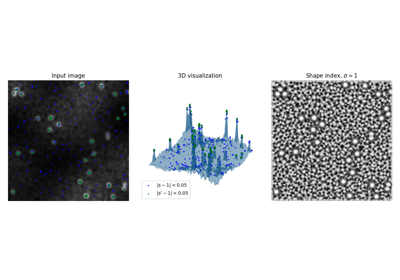## structure_tensor¶

skimage.feature.structure_tensor(image, sigma=1, mode='constant', cval=0, order=None)[source]

Compute structure tensor using sum of squared differences.

The (2-dimensional) structure tensor A is defined as:

A = [Arr Arc]
[Arc Acc]


which is approximated by the weighted sum of squared differences in a local window around each pixel in the image. This formula can be extended to a larger number of dimensions (see ).

Parameters
imagendarray

Input image.

sigmafloat or array-like of float, optional

Standard deviation used for the Gaussian kernel, which is used as a weighting function for the local summation of squared differences. If sigma is an iterable, its length must be equal to image.ndim and each element is used for the Gaussian kernel applied along its respective axis.

mode{‘constant’, ‘reflect’, ‘wrap’, ‘nearest’, ‘mirror’}, optional

How to handle values outside the image borders.

cvalfloat, optional

Used in conjunction with mode ‘constant’, the value outside the image boundaries.

order{‘rc’, ‘xy’}, optional

NOTE: Only applies in 2D. Higher dimensions must always use ‘rc’ order. This parameter allows for the use of reverse or forward order of the image axes in gradient computation. ‘rc’ indicates the use of the first axis initially (Arr, Arc, Acc), whilst ‘xy’ indicates the usage of the last axis initially (Axx, Axy, Ayy).

Returns
A_elemslist of ndarray

Upper-diagonal elements of the structure tensor for each pixel in the input image.

References

1

https://en.wikipedia.org/wiki/Structure_tensor

Examples

>>> from skimage.feature import structure_tensor
>>> square = np.zeros((5, 5))
>>> square[2, 2] = 1
>>> Arr, Arc, Acc = structure_tensor(square, sigma=0.1, order='rc')
>>> Acc
array([[0., 0., 0., 0., 0.],
[0., 1., 0., 1., 0.],
[0., 4., 0., 4., 0.],
[0., 1., 0., 1., 0.],
[0., 0., 0., 0., 0.]])


### Examples using skimage.feature.structure_tensor¶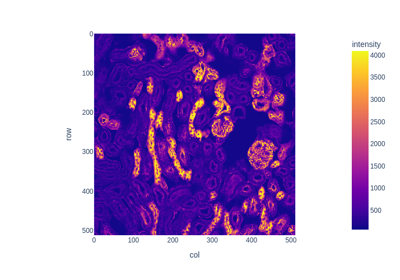## structure_tensor_eigenvalues¶

skimage.feature.structure_tensor_eigenvalues(A_elems)[source]

Compute eigenvalues of structure tensor.

Parameters
A_elemslist of ndarray

The upper-diagonal elements of the structure tensor, as returned by structure_tensor.

Returns
ndarray

The eigenvalues of the structure tensor, in decreasing order. The eigenvalues are the leading dimension. That is, the coordinate [i, j, k] corresponds to the ith-largest eigenvalue at position (j, k).

Examples

>>> from skimage.feature import structure_tensor
>>> from skimage.feature import structure_tensor_eigenvalues
>>> square = np.zeros((5, 5))
>>> square[2, 2] = 1
>>> A_elems = structure_tensor(square, sigma=0.1, order='rc')
>>> structure_tensor_eigenvalues(A_elems)
array([[0., 0., 0., 0., 0.],
[0., 2., 4., 2., 0.],
[0., 4., 0., 4., 0.],
[0., 2., 4., 2., 0.],
[0., 0., 0., 0., 0.]])


### Examples using skimage.feature.structure_tensor_eigenvalues¶## structure_tensor_eigvals¶

skimage.feature.structure_tensor_eigvals(Axx, Axy, Ayy)[source]

Compute eigenvalues of structure tensor.

Parameters
Axxndarray

Element of the structure tensor for each pixel in the input image.

Axyndarray

Element of the structure tensor for each pixel in the input image.

Ayyndarray

Element of the structure tensor for each pixel in the input image.

Returns
l1ndarray

Larger eigen value for each input matrix.

l2ndarray

Smaller eigen value for each input matrix.

Examples

>>> from skimage.feature import structure_tensor, structure_tensor_eigvals
>>> square = np.zeros((5, 5))
>>> square[2, 2] = 1
>>> Arr, Arc, Acc = structure_tensor(square, sigma=0.1, order='rc')
>>> structure_tensor_eigvals(Acc, Arc, Arr)
array([[0., 0., 0., 0., 0.],
[0., 2., 4., 2., 0.],
[0., 4., 0., 4., 0.],
[0., 2., 4., 2., 0.],
[0., 0., 0., 0., 0.]])


## BRIEF¶

class skimage.feature.BRIEF(descriptor_size=256, patch_size=49, mode='normal', sigma=1, sample_seed=1)[source]

Bases: skimage.feature.util.DescriptorExtractor

BRIEF binary descriptor extractor.

BRIEF (Binary Robust Independent Elementary Features) is an efficient feature point descriptor. It is highly discriminative even when using relatively few bits and is computed using simple intensity difference tests.

For each keypoint, intensity comparisons are carried out for a specifically distributed number N of pixel-pairs resulting in a binary descriptor of length N. For binary descriptors the Hamming distance can be used for feature matching, which leads to lower computational cost in comparison to the L2 norm.

Parameters
descriptor_sizeint, optional

Size of BRIEF descriptor for each keypoint. Sizes 128, 256 and 512 recommended by the authors. Default is 256.

patch_sizeint, optional

Length of the two dimensional square patch sampling region around the keypoints. Default is 49.

mode{‘normal’, ‘uniform’}, optional

Probability distribution for sampling location of decision pixel-pairs around keypoints.

sample_seed{None, int, numpy.random.Generator}, optional

If sample_seed is None the numpy.random.Generator singleton is used. If sample_seed is an int, a new Generator instance is used, seeded with sample_seed. If sample_seed is already a Generator instance then that instance is used.

Seed for the random sampling of the decision pixel-pairs. From a square window with length patch_size, pixel pairs are sampled using the mode parameter to build the descriptors using intensity comparison. The value of sample_seed must be the same for the images to be matched while building the descriptors.

sigmafloat, optional

Standard deviation of the Gaussian low-pass filter applied to the image to alleviate noise sensitivity, which is strongly recommended to obtain discriminative and good descriptors.

Examples

>>> from skimage.feature import (corner_harris, corner_peaks, BRIEF,
...                              match_descriptors)
>>> import numpy as np
>>> square1 = np.zeros((8, 8), dtype=np.int32)
>>> square1[2:6, 2:6] = 1
>>> square1
array([[0, 0, 0, 0, 0, 0, 0, 0],
[0, 0, 0, 0, 0, 0, 0, 0],
[0, 0, 1, 1, 1, 1, 0, 0],
[0, 0, 1, 1, 1, 1, 0, 0],
[0, 0, 1, 1, 1, 1, 0, 0],
[0, 0, 1, 1, 1, 1, 0, 0],
[0, 0, 0, 0, 0, 0, 0, 0],
[0, 0, 0, 0, 0, 0, 0, 0]], dtype=int32)
>>> square2 = np.zeros((9, 9), dtype=np.int32)
>>> square2[2:7, 2:7] = 1
>>> square2
array([[0, 0, 0, 0, 0, 0, 0, 0, 0],
[0, 0, 0, 0, 0, 0, 0, 0, 0],
[0, 0, 1, 1, 1, 1, 1, 0, 0],
[0, 0, 1, 1, 1, 1, 1, 0, 0],
[0, 0, 1, 1, 1, 1, 1, 0, 0],
[0, 0, 1, 1, 1, 1, 1, 0, 0],
[0, 0, 1, 1, 1, 1, 1, 0, 0],
[0, 0, 0, 0, 0, 0, 0, 0, 0],
[0, 0, 0, 0, 0, 0, 0, 0, 0]], dtype=int32)
>>> keypoints1 = corner_peaks(corner_harris(square1), min_distance=1)
>>> keypoints2 = corner_peaks(corner_harris(square2), min_distance=1)
>>> extractor = BRIEF(patch_size=5)
>>> extractor.extract(square1, keypoints1)
>>> descriptors1 = extractor.descriptors
>>> extractor.extract(square2, keypoints2)
>>> descriptors2 = extractor.descriptors
>>> matches = match_descriptors(descriptors1, descriptors2)
>>> matches
array([[0, 0],
[1, 1],
[2, 2],
[3, 3]])
>>> keypoints1[matches[:, 0]]
array([[2, 2],
[2, 5],
[5, 2],
[5, 5]])
>>> keypoints2[matches[:, 1]]
array([[2, 2],
[2, 6],
[6, 2],
[6, 6]])

Attributes
descriptors(Q, descriptor_size) array of dtype bool

2D ndarray of binary descriptors of size descriptor_size for Q keypoints after filtering out border keypoints with value at an index (i, j) either being True or False representing the outcome of the intensity comparison for i-th keypoint on j-th decision pixel-pair. It is Q == np.sum(mask).

mask(N, ) array of dtype bool

Mask indicating whether a keypoint has been filtered out (False) or is described in the descriptors array (True).

__init__(descriptor_size=256, patch_size=49, mode='normal', sigma=1, sample_seed=1)[source]

Initialize self. See help(type(self)) for accurate signature.

extract(image, keypoints)[source]

Extract BRIEF binary descriptors for given keypoints in image.

Parameters
image2D array

Input image.

keypoints(N, 2) array

Keypoint coordinates as (row, col).

### Examples using skimage.feature.BRIEF¶## CENSURE¶

class skimage.feature.CENSURE(min_scale=1, max_scale=7, mode='DoB', non_max_threshold=0.15, line_threshold=10)[source]

Bases: skimage.feature.util.FeatureDetector

CENSURE keypoint detector.

min_scaleint, optional

Minimum scale to extract keypoints from.

max_scaleint, optional

Maximum scale to extract keypoints from. The keypoints will be extracted from all the scales except the first and the last i.e. from the scales in the range [min_scale + 1, max_scale - 1]. The filter sizes for different scales is such that the two adjacent scales comprise of an octave.

mode{‘DoB’, ‘Octagon’, ‘STAR’}, optional

Type of bi-level filter used to get the scales of the input image. Possible values are ‘DoB’, ‘Octagon’ and ‘STAR’. The three modes represent the shape of the bi-level filters i.e. box(square), octagon and star respectively. For instance, a bi-level octagon filter consists of a smaller inner octagon and a larger outer octagon with the filter weights being uniformly negative in both the inner octagon while uniformly positive in the difference region. Use STAR and Octagon for better features and DoB for better performance.

non_max_thresholdfloat, optional

Threshold value used to suppress maximas and minimas with a weak magnitude response obtained after Non-Maximal Suppression.

line_thresholdfloat, optional

Threshold for rejecting interest points which have ratio of principal curvatures greater than this value.

References

1

Motilal Agrawal, Kurt Konolige and Morten Rufus Blas “CENSURE: Center Surround Extremas for Realtime Feature Detection and Matching”, https://link.springer.com/chapter/10.1007/978-3-540-88693-8_8 DOI:10.1007/978-3-540-88693-8_8

2

Adam Schmidt, Marek Kraft, Michal Fularz and Zuzanna Domagala “Comparative Assessment of Point Feature Detectors and Descriptors in the Context of Robot Navigation” http://yadda.icm.edu.pl/yadda/element/bwmeta1.element.baztech-268aaf28-0faf-4872-a4df-7e2e61cb364c/c/Schmidt_comparative.pdf DOI:10.1.1.465.1117

Examples

>>> from skimage.data import astronaut
>>> from skimage.color import rgb2gray
>>> from skimage.feature import CENSURE
>>> img = rgb2gray(astronaut()[100:300, 100:300])
>>> censure = CENSURE()
>>> censure.detect(img)
>>> censure.keypoints
array([[  4, 148],
[ 12,  73],
[ 21, 176],
[ 91,  22],
[ 93,  56],
[ 94,  22],
[ 95,  54],
[100,  51],
[103,  51],
[106,  67],
[108,  15],
[117,  20],
[122,  60],
[125,  37],
[129,  37],
[133,  76],
[145,  44],
[146,  94],
[150, 114],
[153,  33],
[154, 156],
[155, 151],
[184,  63]])
>>> censure.scales
array([2, 6, 6, 2, 4, 3, 2, 3, 2, 6, 3, 2, 2, 3, 2, 2, 2, 3, 2, 2, 4, 2,
2])

Attributes
keypoints(N, 2) array

Keypoint coordinates as (row, col).

scales(N, ) array

Corresponding scales.

__init__(min_scale=1, max_scale=7, mode='DoB', non_max_threshold=0.15, line_threshold=10)[source]

Initialize self. See help(type(self)) for accurate signature.

detect(image)[source]

Detect CENSURE keypoints along with the corresponding scale.

Parameters
image2D ndarray

Input image.

### Examples using skimage.feature.CENSURE¶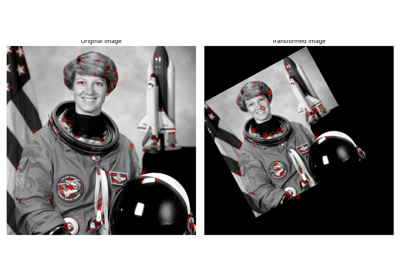## Cascade¶

class skimage.feature.Cascade

Bases: object

Class for cascade of classifiers that is used for object detection.

The main idea behind cascade of classifiers is to create classifiers of medium accuracy and ensemble them into one strong classifier instead of just creating a strong one. The second advantage of cascade classifier is that easy examples can be classified only by evaluating some of the classifiers in the cascade, making the process much faster than the process of evaluating a one strong classifier.

Notes

The cascade approach was first described by Viola and Jones , , although these initial publications used a set of Haar-like features. This implementation instead uses multi-scale block local binary pattern (MB-LBP) features .

References

1

Viola, P. and Jones, M. “Rapid object detection using a boosted cascade of simple features,” In: Proceedings of the 2001 IEEE Computer Society Conference on Computer Vision and Pattern Recognition. CVPR 2001, pp. I-I. DOI:10.1109/CVPR.2001.990517

2

Viola, P. and Jones, M.J, “Robust Real-Time Face Detection”, International Journal of Computer Vision 57, 137–154 (2004). DOI:10.1023/B:VISI.0000013087.49260.fb

3

Liao, S. et al. Learning Multi-scale Block Local Binary Patterns for Face Recognition. International Conference on Biometrics (ICB), 2007, pp. 828-837. In: Lecture Notes in Computer Science, vol 4642. Springer, Berlin, Heidelberg. DOI:10.1007/978-3-540-74549-5_87

Attributes
epscnp.float32_t

Accuracy parameter. Increasing it, makes the classifier detect less false positives but at the same time the false negative score increases.

stages_numberPy_ssize_t

Amount of stages in a cascade. Each cascade consists of stumps i.e. trained features.

stumps_numberPy_ssize_t

The overall amount of stumps in all the stages of cascade.

features_numberPy_ssize_t

The overall amount of different features used by cascade. Two stumps can use the same features but has different trained values.

window_widthPy_ssize_t

The width of a detection window that is used. Objects smaller than this window can’t be detected.

window_heightPy_ssize_t

The height of a detection window.

stagesStage*

A pointer to the C array that stores stages information using a Stage struct.

featuresMBLBP*

A pointer to the C array that stores MBLBP features using an MBLBP struct.

LUTscnp.uint32_t*

A pointer to the C array with look-up tables that are used by trained MBLBP features (MBLBPStumps) to evaluate a particular region.

__init__()

Parameters
xml_filefile’s path or file’s object

A file in a OpenCv format from which all the cascade classifier’s parameters are loaded.

epscnp.float32_t

Accuracy parameter. Increasing it, makes the classifier detect less false positives but at the same time the false negative score increases.

detect_multi_scale()

Search for the object on multiple scales of input image.

The function takes the input image, the scale factor by which the searching window is multiplied on each step, minimum window size and maximum window size that specify the interval for the search windows that are applied to the input image to detect objects.

Parameters
img2-D or 3-D ndarray

Ndarray that represents the input image.

scale_factorcnp.float32_t

The scale by which searching window is multiplied on each step.

step_ratiocnp.float32_t

The ratio by which the search step in multiplied on each scale of the image. 1 represents the exaustive search and usually is slow. By setting this parameter to higher values the results will be worse but the computation will be much faster. Usually, values in the interval [1, 1.5] give good results.

min_sizetyple (int, int)

Minimum size of the search window.

max_sizetyple (int, int)

Maximum size of the search window.

min_neighbour_numberint

Minimum amount of intersecting detections in order for detection to be approved by the function.

intersection_score_thresholdcnp.float32_t

The minimum value of value of ratio (intersection area) / (small rectangle ratio) in order to merge two detections into one.

Returns
outputlist of dicts

Dict have form {‘r’: int, ‘c’: int, ‘width’: int, ‘height’: int}, where ‘r’ represents row position of top left corner of detected window, ‘c’ - col position, ‘width’ - width of detected window, ‘height’ - height of detected window.

eps
features_number
stages_number
stumps_number
window_height
window_width

### Examples using skimage.feature.Cascade¶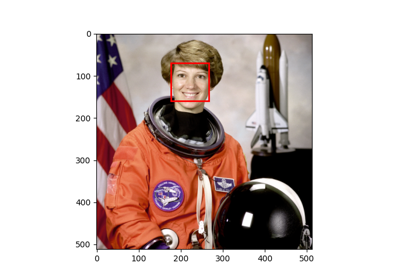## ORB¶

class skimage.feature.ORB(downscale=1.2, n_scales=8, n_keypoints=500, fast_n=9, fast_threshold=0.08, harris_k=0.04)[source]

Bases: skimage.feature.util.FeatureDetector, skimage.feature.util.DescriptorExtractor

Oriented FAST and rotated BRIEF feature detector and binary descriptor extractor.

Parameters
n_keypointsint, optional

Number of keypoints to be returned. The function will return the best n_keypoints according to the Harris corner response if more than n_keypoints are detected. If not, then all the detected keypoints are returned.

fast_nint, optional

The n parameter in skimage.feature.corner_fast. Minimum number of consecutive pixels out of 16 pixels on the circle that should all be either brighter or darker w.r.t test-pixel. A point c on the circle is darker w.r.t test pixel p if Ic < Ip - threshold and brighter if Ic > Ip + threshold. Also stands for the n in FAST-n corner detector.

fast_thresholdfloat, optional

The threshold parameter in feature.corner_fast. Threshold used to decide whether the pixels on the circle are brighter, darker or similar w.r.t. the test pixel. Decrease the threshold when more corners are desired and vice-versa.

harris_kfloat, optional

The k parameter in skimage.feature.corner_harris. Sensitivity factor to separate corners from edges, typically in range [0, 0.2]. Small values of k result in detection of sharp corners.

downscalefloat, optional

Downscale factor for the image pyramid. Default value 1.2 is chosen so that there are more dense scales which enable robust scale invariance for a subsequent feature description.

n_scalesint, optional

Maximum number of scales from the bottom of the image pyramid to extract the features from.

References

1

Ethan Rublee, Vincent Rabaud, Kurt Konolige and Gary Bradski “ORB: An efficient alternative to SIFT and SURF” http://www.vision.cs.chubu.ac.jp/CV-R/pdf/Rublee_iccv2011.pdf

Examples

>>> from skimage.feature import ORB, match_descriptors
>>> img1 = np.zeros((100, 100))
>>> img2 = np.zeros_like(img1)
>>> rng = np.random.default_rng(19481137)  # do not copy this value
>>> square = rng.random((20, 20))
>>> img1[40:60, 40:60] = square
>>> img2[53:73, 53:73] = square
>>> detector_extractor1 = ORB(n_keypoints=5)
>>> detector_extractor2 = ORB(n_keypoints=5)
>>> detector_extractor1.detect_and_extract(img1)
>>> detector_extractor2.detect_and_extract(img2)
>>> matches = match_descriptors(detector_extractor1.descriptors,
...                             detector_extractor2.descriptors)
>>> matches
array([[0, 0],
[1, 1],
[2, 2],
[3, 4],
[4, 3]])
>>> detector_extractor1.keypoints[matches[:, 0]]
array([[59. , 59. ],
[40. , 40. ],
[57. , 40. ],
[46. , 58. ],
[58.8, 58.8]])
>>> detector_extractor2.keypoints[matches[:, 1]]
array([[72., 72.],
[53., 53.],
[70., 53.],
[59., 71.],
[72., 72.]])

Attributes
keypoints(N, 2) array

Keypoint coordinates as (row, col).

scales(N, ) array

Corresponding scales.

orientations(N, ) array

responses(N, ) array

Corresponding Harris corner responses.

descriptors(Q, descriptor_size) array of dtype bool

2D array of binary descriptors of size descriptor_size for Q keypoints after filtering out border keypoints with value at an index (i, j) either being True or False representing the outcome of the intensity comparison for i-th keypoint on j-th decision pixel-pair. It is Q == np.sum(mask).

__init__(downscale=1.2, n_scales=8, n_keypoints=500, fast_n=9, fast_threshold=0.08, harris_k=0.04)[source]

Initialize self. See help(type(self)) for accurate signature.

detect(image)[source]

Detect oriented FAST keypoints along with the corresponding scale.

Parameters
image2D array

Input image.

detect_and_extract(image)[source]

Detect oriented FAST keypoints and extract rBRIEF descriptors.

Note that this is faster than first calling detect and then extract.

Parameters
image2D array

Input image.

extract(image, keypoints, scales, orientations)[source]

Extract rBRIEF binary descriptors for given keypoints in image.

Note that the keypoints must be extracted using the same downscale and n_scales parameters. Additionally, if you want to extract both keypoints and descriptors you should use the faster detect_and_extract.

Parameters
image2D array

Input image.

keypoints(N, 2) array

Keypoint coordinates as (row, col).

scales(N, ) array

Corresponding scales.

orientations(N, ) array

### Examples using skimage.feature.ORB¶## SIFT¶

class skimage.feature.SIFT(upsampling=2, n_octaves=8, n_scales=3, sigma_min=1.6, sigma_in=0.5, c_dog=0.013333333333333334, c_edge=10, n_bins=36, lambda_ori=1.5, c_max=0.8, lambda_descr=6, n_hist=4, n_ori=8)[source]

Bases: skimage.feature.util.FeatureDetector, skimage.feature.util.DescriptorExtractor

SIFT feature detection and descriptor extraction.

Parameters
upsamplingint, optional

Prior to the feature detection the image is upscaled by a factor of 1 (no upscaling), 2 or 4. Method: Bi-cubic interpolation.

n_octavesint, optional

Maximum number of octaves. With every octave the image size is halved and the sigma doubled. The number of octaves will be reduced as needed to keep at least 12 pixels along each dimension at the smallest scale.

n_scalesint, optional

Maximum number of scales in every octave.

sigma_minfloat, optional

The blur level of the seed image. If upsampling is enabled sigma_min is scaled by factor 1/upsampling

sigma_infloat, optional

The assumed blur level of the input image.

c_dogfloat, optional

Threshold to discard low contrast extrema in the DoG. It’s final value is dependent on n_scales by the relation: final_c_dog = (2^(1/n_scales)-1) / (2^(1/3)-1) * c_dog

c_edgefloat, optional

Threshold to discard extrema that lie in edges. If H is the Hessian of an extremum, its “edgeness” is described by tr(H)²/det(H). If the edgeness is higher than (c_edge + 1)²/c_edge, the extremum is discarded.

n_binsint, optional

Number of bins in the histogram that describes the gradient orientations around keypoint.

lambda_orifloat, optional

The window used to find the reference orientation of a keypoint has a width of 6 * lambda_ori * sigma and is weighted by a standard deviation of 2 * lambda_ori * sigma.

c_maxfloat, optional

The threshold at which a secondary peak in the orientation histogram is accepted as orientation

lambda_descrfloat, optional

The window used to define the descriptor of a keypoint has a width of 2 * lambda_descr * sigma * (n_hist+1)/n_hist and is weighted by a standard deviation of lambda_descr * sigma.

n_histint, optional

The window used to define the descriptor of a keypoint consists of n_hist * n_hist histograms.

n_oriint, optional

The number of bins in the histograms of the descriptor patch.

Notes

The SIFT algorithm was developed by David Lowe ,  and later patented by the University of British Columbia. Since the patent expired in 2020 it’s free to use. The implementation here closely follows the detailed description in , including use of the same default parameters.

References

1

D.G. Lowe. “Object recognition from local scale-invariant features”, Proceedings of the Seventh IEEE International Conference on Computer Vision, 1999, vol.2, pp. 1150-1157. DOI:10.1109/ICCV.1999.790410

2

D.G. Lowe. “Distinctive Image Features from Scale-Invariant Keypoints”, International Journal of Computer Vision, 2004, vol. 60, pp. 91–110. DOI:10.1023/B:VISI.0000029664.99615.94

3

I. R. Otero and M. Delbracio. “Anatomy of the SIFT Method”, Image Processing On Line, 4 (2014), pp. 370–396. DOI:10.5201/ipol.2014.82

Examples

>>> from skimage.feature import SIFT, match_descriptors
>>> from skimage.data import camera
>>> from skimage.transform import rotate
>>> img1 = camera()
>>> img2 = rotate(camera(), 90)
>>> detector_extractor1 = SIFT()
>>> detector_extractor2 = SIFT()
>>> detector_extractor1.detect_and_extract(img1)
>>> detector_extractor2.detect_and_extract(img2)
>>> matches = match_descriptors(detector_extractor1.descriptors,
...                             detector_extractor2.descriptors,
...                             max_ratio=0.6)
>>> matches[10:15]
array([[ 10, 412],
[ 11, 417],
[ 12, 407],
[ 13, 411],
[ 14, 406]])
>>> detector_extractor1.keypoints[matches[10:15, 0]]
array([[ 95, 214],
[ 97, 211],
[ 97, 218],
[102, 215],
[104, 218]])
>>> detector_extractor2.keypoints[matches[10:15, 1]]
array([[297,  95],
[301,  97],
[294,  97],
[297, 102],
[293, 104]])

Attributes
delta_minfloat

The sampling distance of the first octave. It’s final value is 1/upsampling.

float_dtypetype

The datatype of the image.

scalespace_sigmas(n_octaves, n_scales + 3) array

The sigma value of all scales in all octaves.

keypoints(N, 2) array

Keypoint coordinates as (row, col).

positions(N, 2) array

Subpixel-precision keypoint coordinates as (row, col).

sigmas(N, ) array

The corresponding sigma (blur) value of a keypoint.

scales(N, ) array

The corresponding scale of a keypoint.

orientations(N, ) array

The orientations of the gradient around every keypoint.

octaves(N, ) array

The corresponding octave of a keypoint.

descriptors(N, n_hist*n_hist*n_ori) array

The descriptors of a keypoint.

__init__(upsampling=2, n_octaves=8, n_scales=3, sigma_min=1.6, sigma_in=0.5, c_dog=0.013333333333333334, c_edge=10, n_bins=36, lambda_ori=1.5, c_max=0.8, lambda_descr=6, n_hist=4, n_ori=8)[source]

Initialize self. See help(type(self)) for accurate signature.

property deltas

The sampling distances of all octaves

detect(image)[source]

Detect the keypoints.

Parameters
image2D array

Input image.

detect_and_extract(image)[source]

Detect the keypoints and extract their descriptors.

Parameters
image2D array

Input image.

extract(image)[source]

Extract the descriptors for all keypoints in the image.

Parameters
image2D array

Input image.

### Examples using skimage.feature.SIFT¶